Скачать презентацию Chapter 4 Preview Objectives Properties of

e9c6ecc8945697671a392e76c45906d2.ppt

• Количество слайдов: 87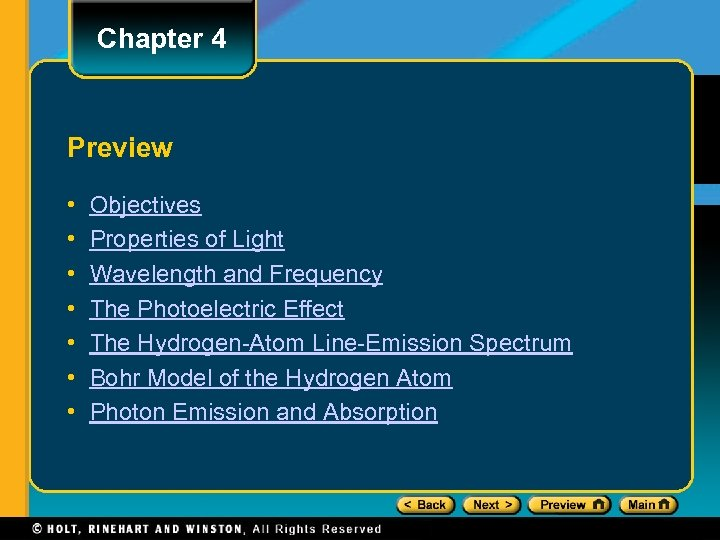Chapter 4 Preview • • Objectives Properties of Light Wavelength and Frequency The Photoelectric Effect The Hydrogen-Atom Line-Emission Spectrum Bohr Model of the Hydrogen Atom Photon Emission and Absorption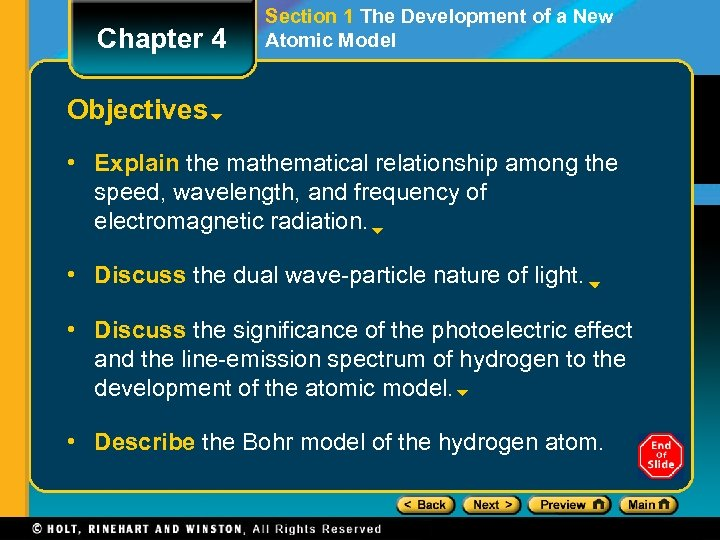Chapter 4 Section 1 The Development of a New Atomic Model Objectives • Explain the mathematical relationship among the speed, wavelength, and frequency of electromagnetic radiation. • Discuss the dual wave-particle nature of light. • Discuss the significance of the photoelectric effect and the line-emission spectrum of hydrogen to the development of the atomic model. • Describe the Bohr model of the hydrogen atom.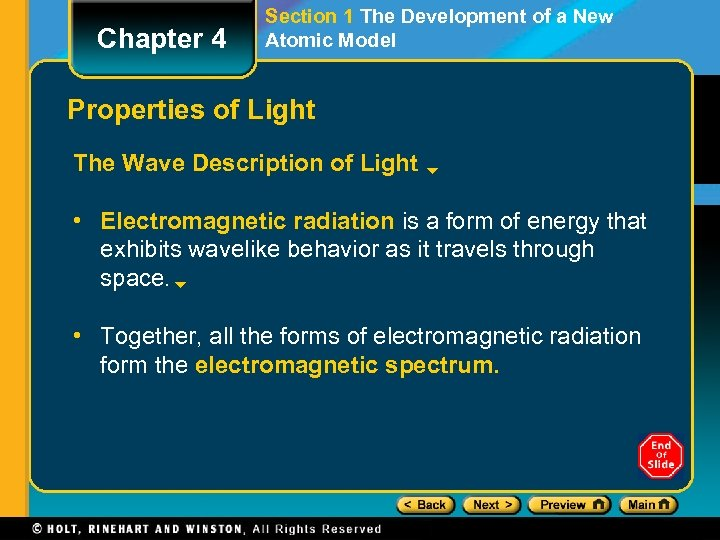Chapter 4 Section 1 The Development of a New Atomic Model Properties of Light The Wave Description of Light • Electromagnetic radiation is a form of energy that exhibits wavelike behavior as it travels through space. • Together, all the forms of electromagnetic radiation form the electromagnetic spectrum.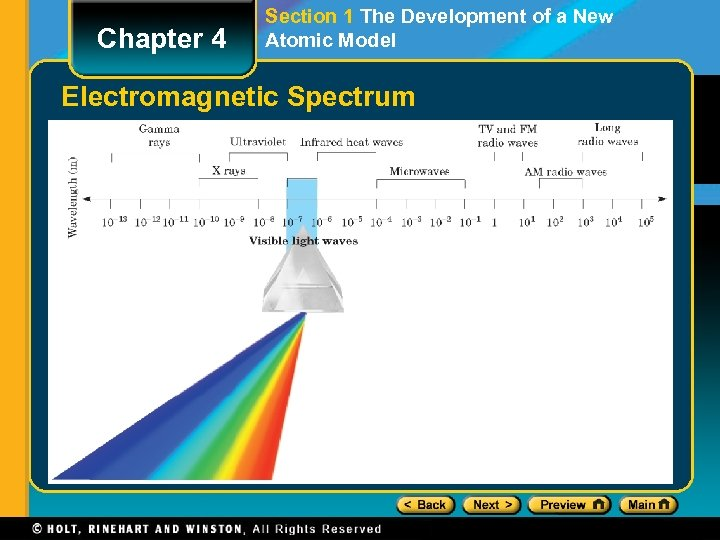Chapter 4 Section 1 The Development of a New Atomic Model Electromagnetic Spectrum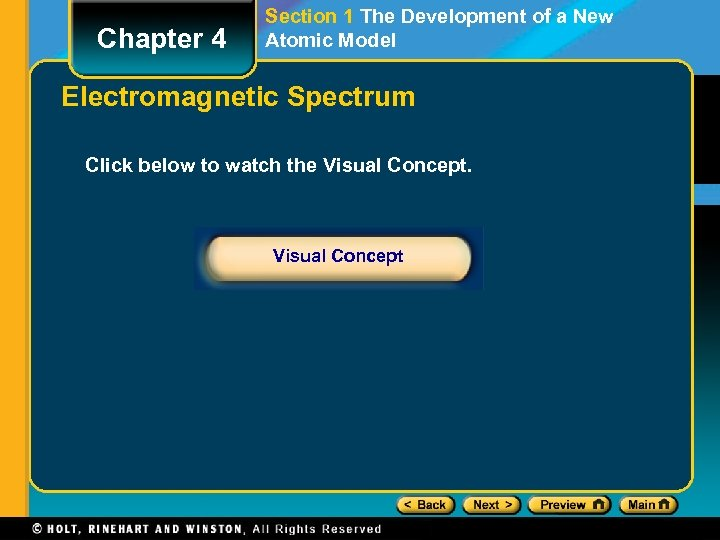Chapter 4 Section 1 The Development of a New Atomic Model Electromagnetic Spectrum Click below to watch the Visual Concept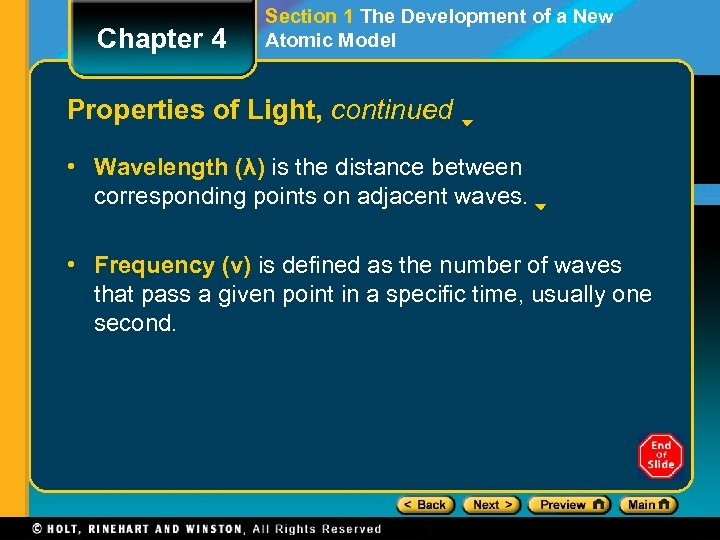Chapter 4 Section 1 The Development of a New Atomic Model Properties of Light, continued • Wavelength (λ) is the distance between corresponding points on adjacent waves. • Frequency (ν) is defined as the number of waves that pass a given point in a specific time, usually one second.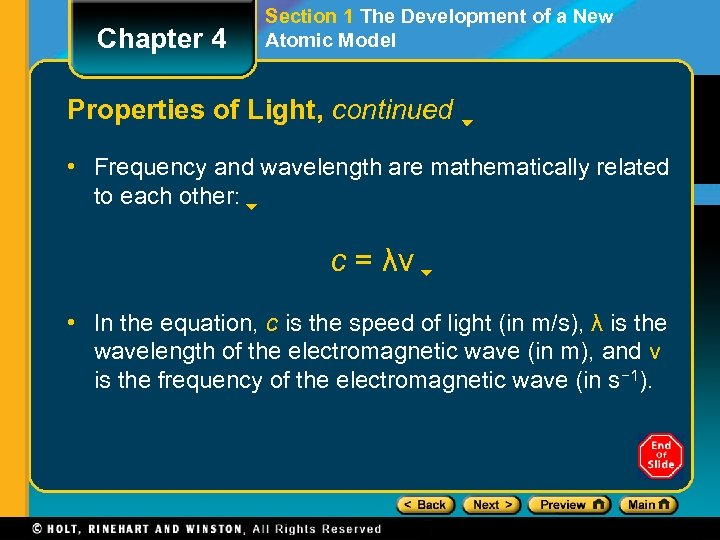Chapter 4 Section 1 The Development of a New Atomic Model Properties of Light, continued • Frequency and wavelength are mathematically related to each other: c = λν • In the equation, c is the speed of light (in m/s), λ is the wavelength of the electromagnetic wave (in m), and ν is the frequency of the electromagnetic wave (in s− 1).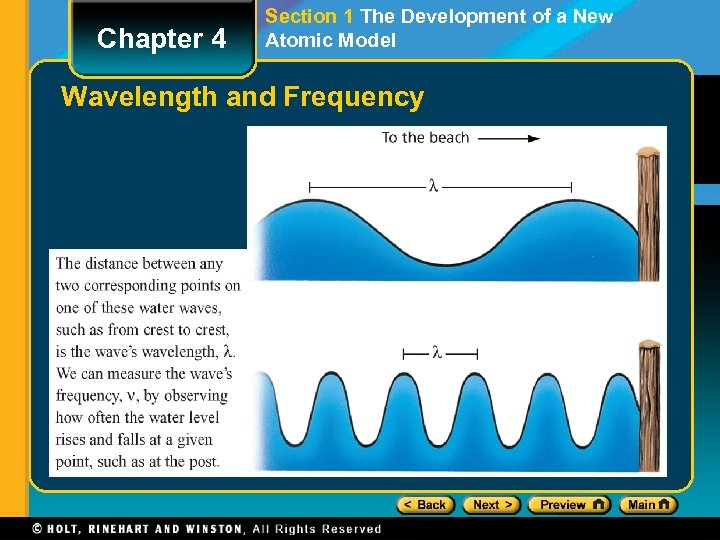Chapter 4 Section 1 The Development of a New Atomic Model Wavelength and Frequency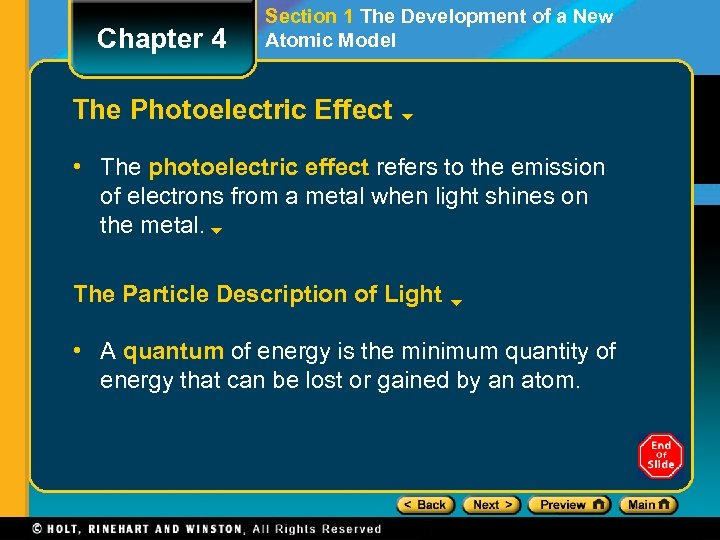Chapter 4 Section 1 The Development of a New Atomic Model The Photoelectric Effect • The photoelectric effect refers to the emission of electrons from a metal when light shines on the metal. The Particle Description of Light • A quantum of energy is the minimum quantity of energy that can be lost or gained by an atom.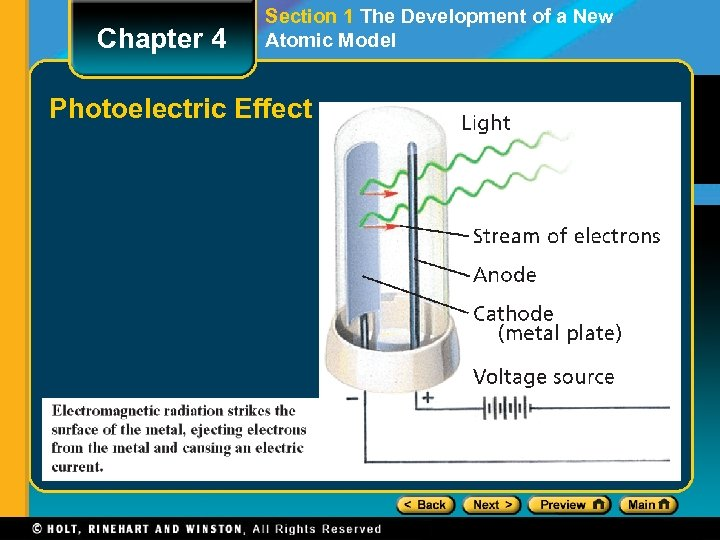Chapter 4 Section 1 The Development of a New Atomic Model Photoelectric Effect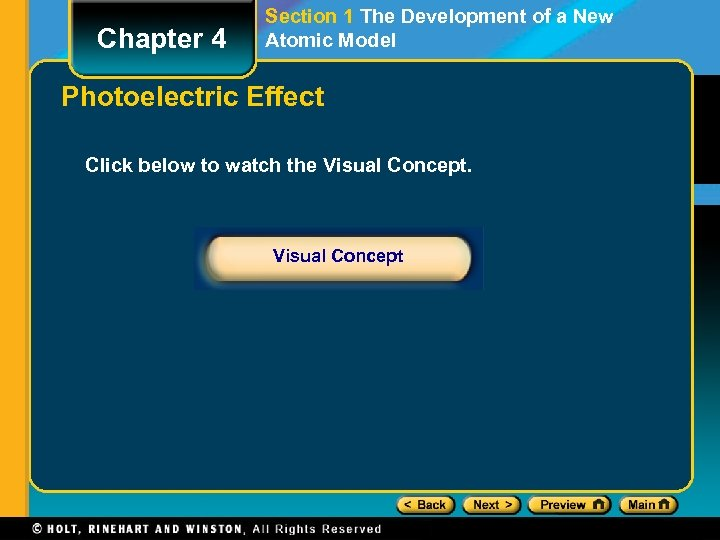Chapter 4 Section 1 The Development of a New Atomic Model Photoelectric Effect Click below to watch the Visual Concept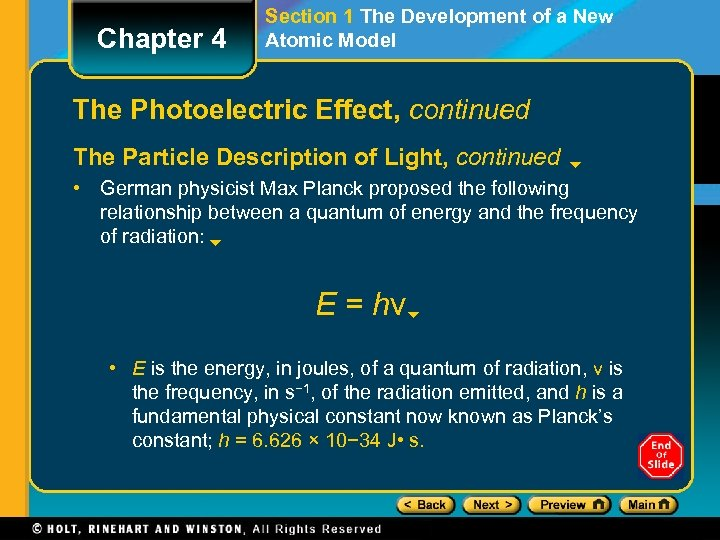Chapter 4 Section 1 The Development of a New Atomic Model The Photoelectric Effect, continued The Particle Description of Light, continued • German physicist Max Planck proposed the following relationship between a quantum of energy and the frequency of radiation: E = hν • E is the energy, in joules, of a quantum of radiation, ν is the frequency, in s− 1, of the radiation emitted, and h is a fundamental physical constant now known as Planck’s constant; h = 6. 626 × 10− 34 J • s.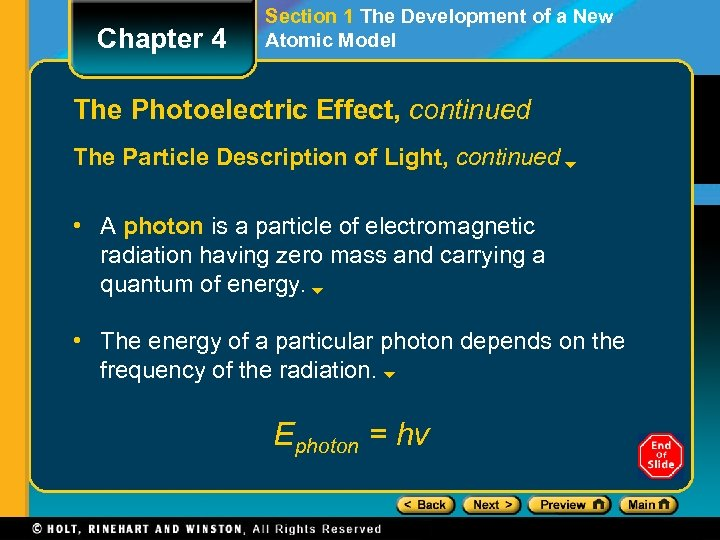Chapter 4 Section 1 The Development of a New Atomic Model The Photoelectric Effect, continued The Particle Description of Light, continued • A photon is a particle of electromagnetic radiation having zero mass and carrying a quantum of energy. • The energy of a particular photon depends on the frequency of the radiation. Ephoton = hν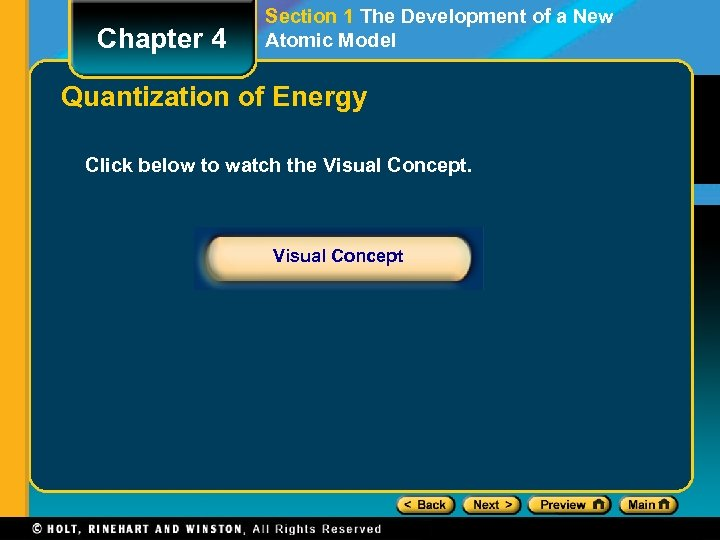Chapter 4 Section 1 The Development of a New Atomic Model Quantization of Energy Click below to watch the Visual Concept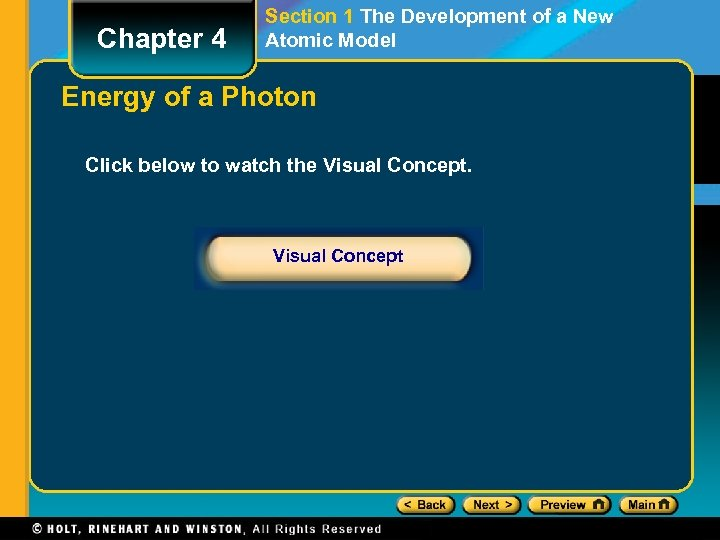Chapter 4 Section 1 The Development of a New Atomic Model Energy of a Photon Click below to watch the Visual Concept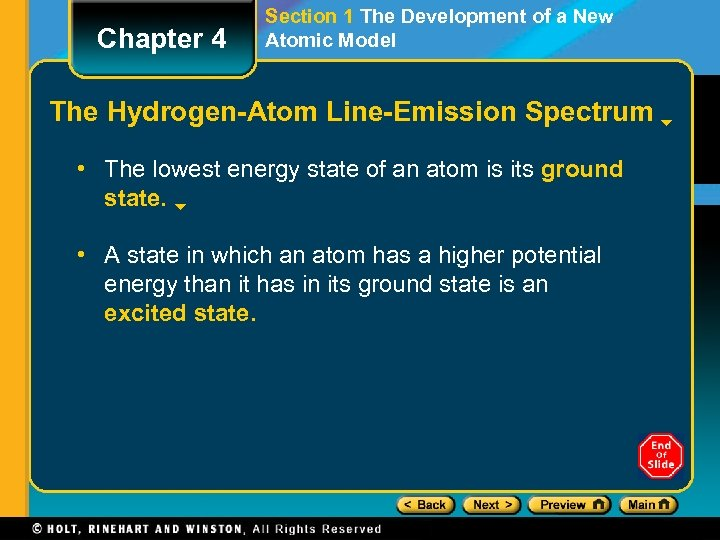Chapter 4 Section 1 The Development of a New Atomic Model The Hydrogen-Atom Line-Emission Spectrum • The lowest energy state of an atom is its ground state. • A state in which an atom has a higher potential energy than it has in its ground state is an excited state.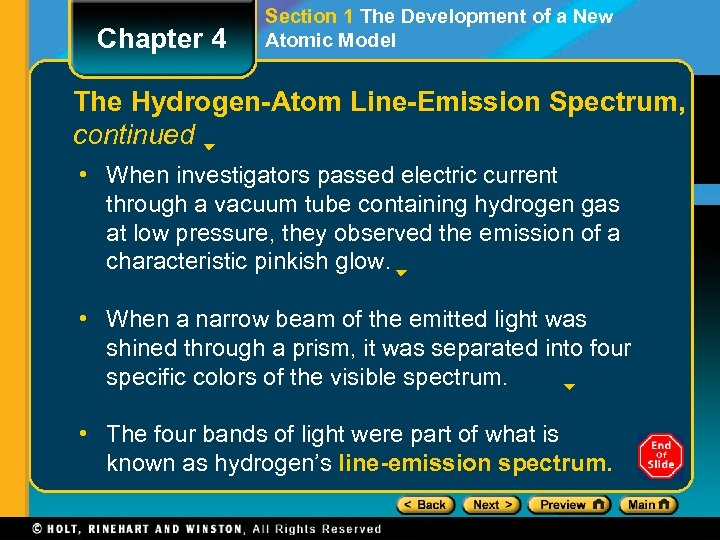Chapter 4 Section 1 The Development of a New Atomic Model The Hydrogen-Atom Line-Emission Spectrum, continued • When investigators passed electric current through a vacuum tube containing hydrogen gas at low pressure, they observed the emission of a characteristic pinkish glow. • When a narrow beam of the emitted light was shined through a prism, it was separated into four specific colors of the visible spectrum. • The four bands of light were part of what is known as hydrogen’s line-emission spectrum.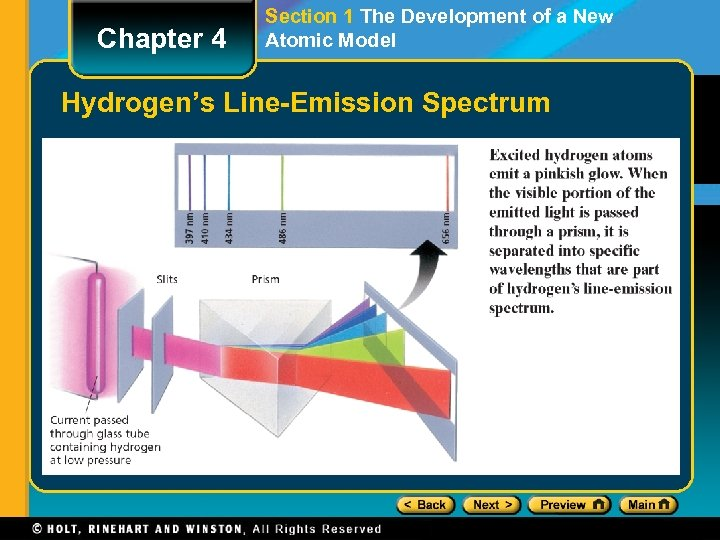Chapter 4 Section 1 The Development of a New Atomic Model Hydrogen’s Line-Emission SpectrumChapter 4 Section 1 The Development of a New Atomic Model Absorption and Emission Spectra Click below to watch the Visual Concept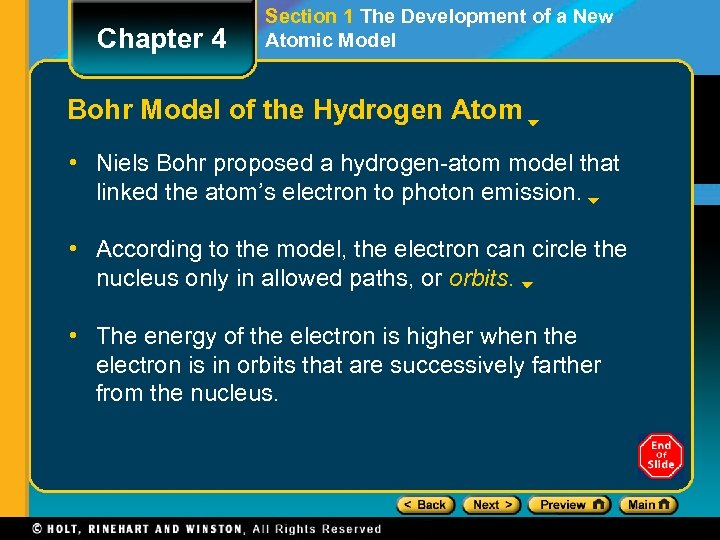Chapter 4 Section 1 The Development of a New Atomic Model Bohr Model of the Hydrogen Atom • Niels Bohr proposed a hydrogen-atom model that linked the atom’s electron to photon emission. • According to the model, the electron can circle the nucleus only in allowed paths, or orbits. • The energy of the electron is higher when the electron is in orbits that are successively farther from the nucleus.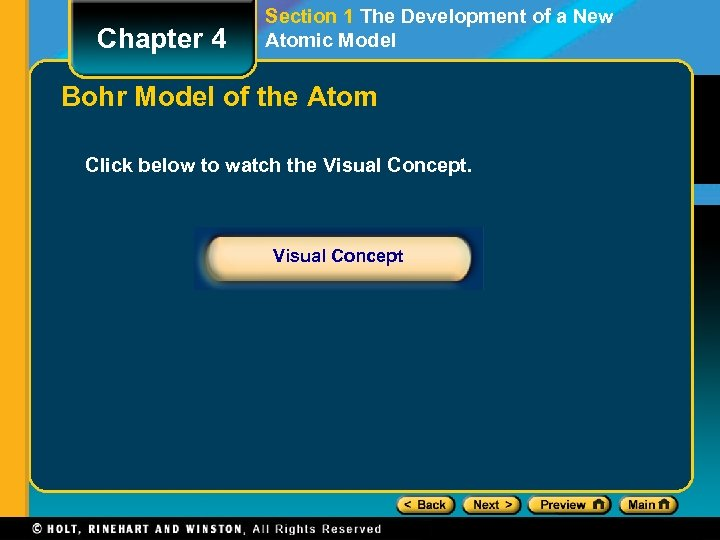Chapter 4 Section 1 The Development of a New Atomic Model Bohr Model of the Atom Click below to watch the Visual Concept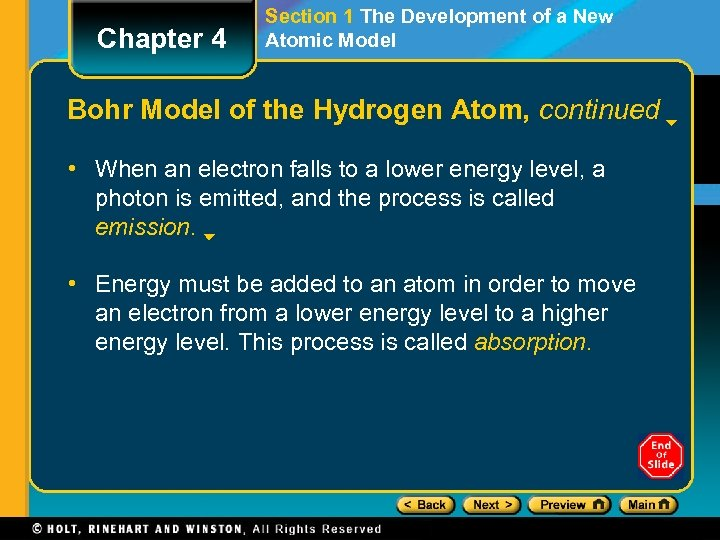Chapter 4 Section 1 The Development of a New Atomic Model Bohr Model of the Hydrogen Atom, continued • When an electron falls to a lower energy level, a photon is emitted, and the process is called emission. • Energy must be added to an atom in order to move an electron from a lower energy level to a higher energy level. This process is called absorption.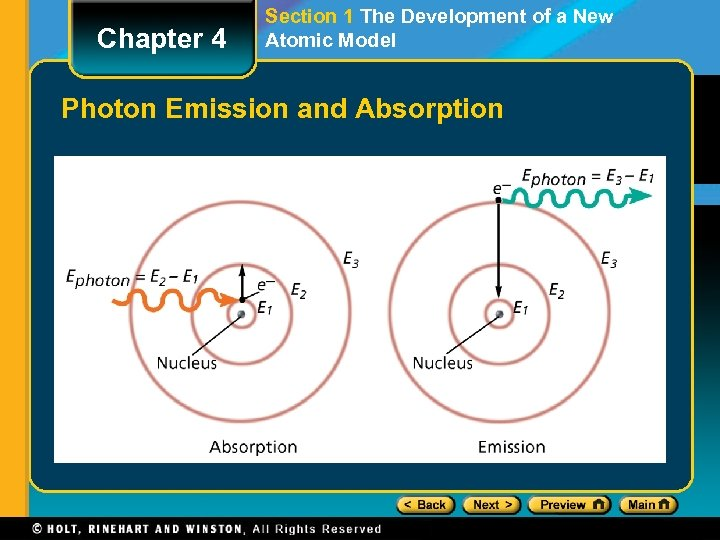Chapter 4 Section 1 The Development of a New Atomic Model Photon Emission and Absorption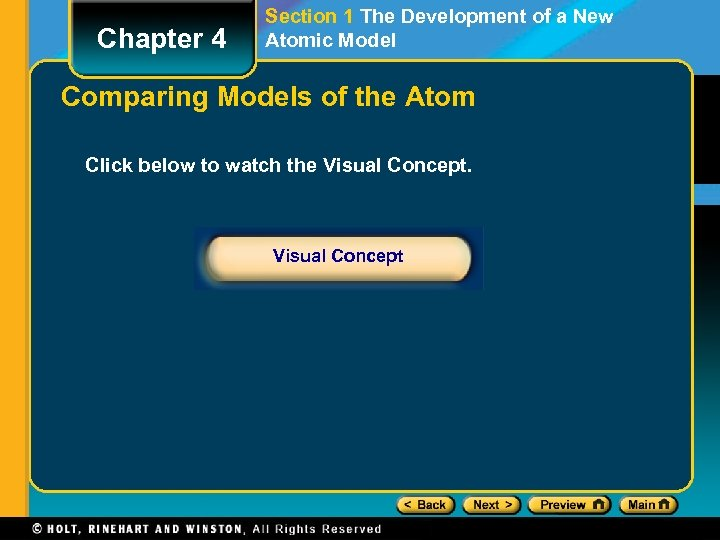Chapter 4 Section 1 The Development of a New Atomic Model Comparing Models of the Atom Click below to watch the Visual Concept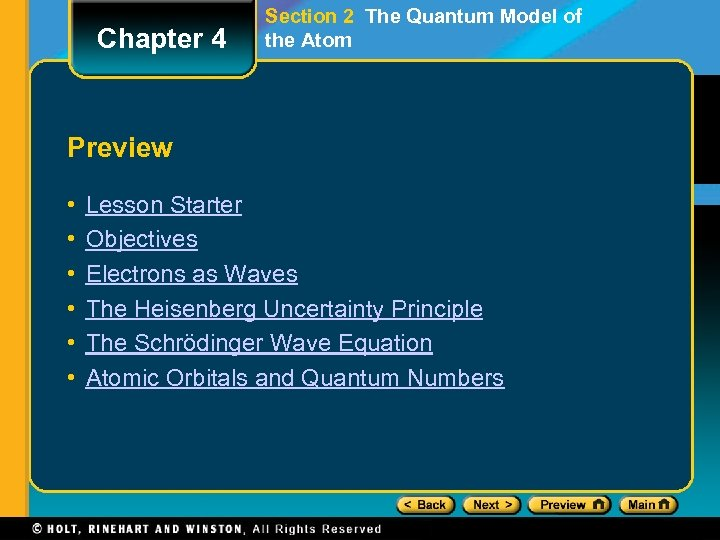Chapter 4 Section 2 The Quantum Model of the Atom Preview • • • Lesson Starter Objectives Electrons as Waves The Heisenberg Uncertainty Principle The Schrödinger Wave Equation Atomic Orbitals and Quantum Numbers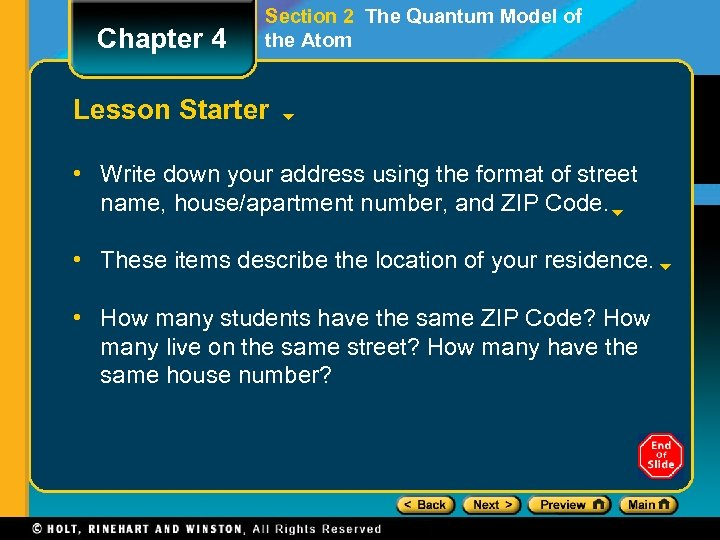Chapter 4 Section 2 The Quantum Model of the Atom Lesson Starter • Write down your address using the format of street name, house/apartment number, and ZIP Code. • These items describe the location of your residence. • How many students have the same ZIP Code? How many live on the same street? How many have the same house number?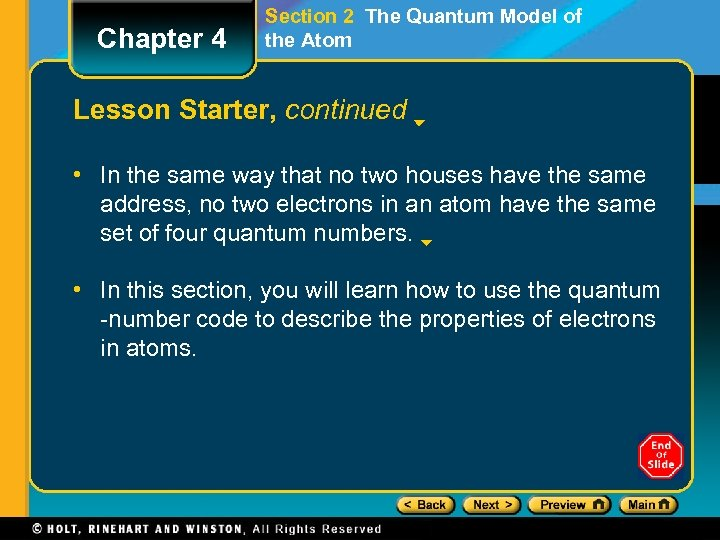Chapter 4 Section 2 The Quantum Model of the Atom Lesson Starter, continued • In the same way that no two houses have the same address, no two electrons in an atom have the same set of four quantum numbers. • In this section, you will learn how to use the quantum -number code to describe the properties of electrons in atoms.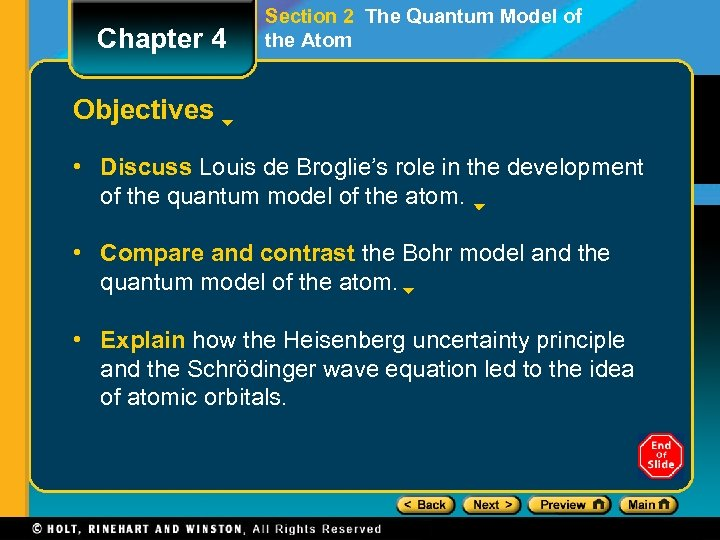Chapter 4 Section 2 The Quantum Model of the Atom Objectives • Discuss Louis de Broglie’s role in the development of the quantum model of the atom. • Compare and contrast the Bohr model and the quantum model of the atom. • Explain how the Heisenberg uncertainty principle and the Schrödinger wave equation led to the idea of atomic orbitals.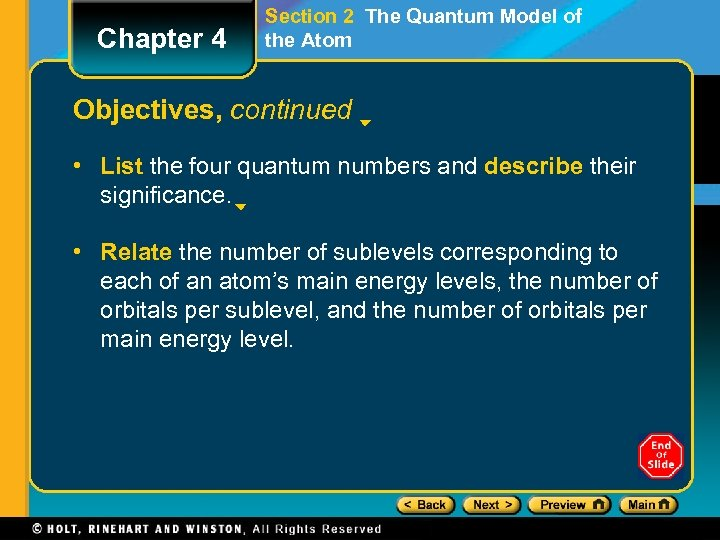Chapter 4 Section 2 The Quantum Model of the Atom Objectives, continued • List the four quantum numbers and describe their significance. • Relate the number of sublevels corresponding to each of an atom’s main energy levels, the number of orbitals per sublevel, and the number of orbitals per main energy level.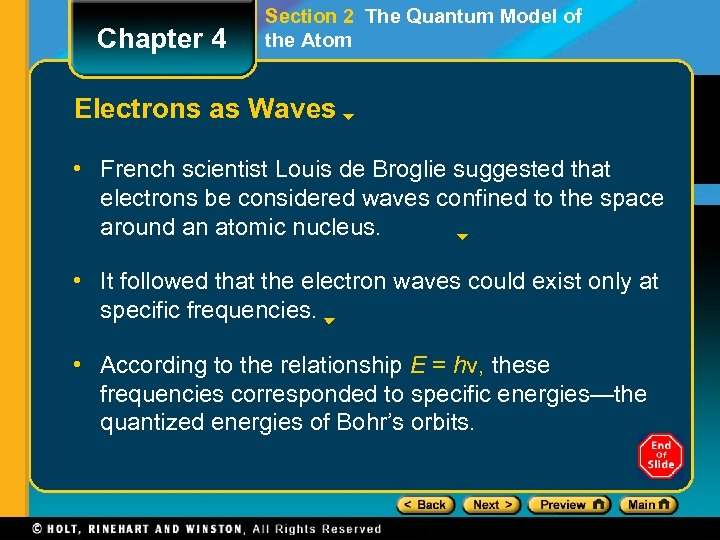Chapter 4 Section 2 The Quantum Model of the Atom Electrons as Waves • French scientist Louis de Broglie suggested that electrons be considered waves confined to the space around an atomic nucleus. • It followed that the electron waves could exist only at specific frequencies. • According to the relationship E = hν, these frequencies corresponded to specific energies—the quantized energies of Bohr’s orbits.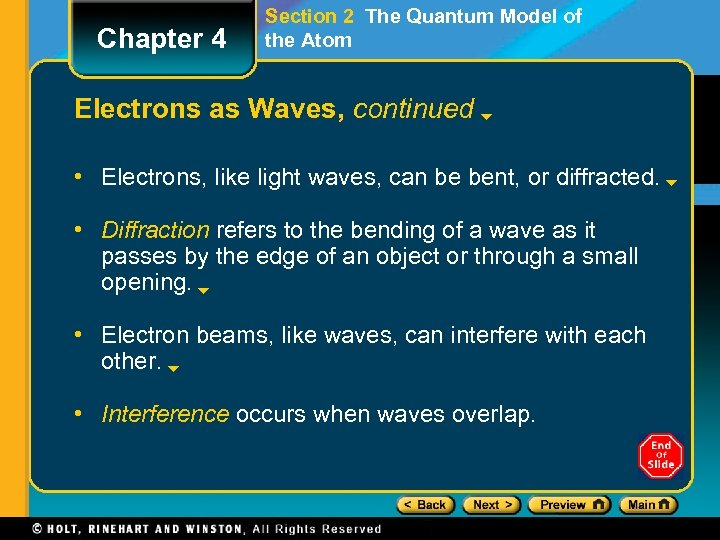Chapter 4 Section 2 The Quantum Model of the Atom Electrons as Waves, continued • Electrons, like light waves, can be bent, or diffracted. • Diffraction refers to the bending of a wave as it passes by the edge of an object or through a small opening. • Electron beams, like waves, can interfere with each other. • Interference occurs when waves overlap.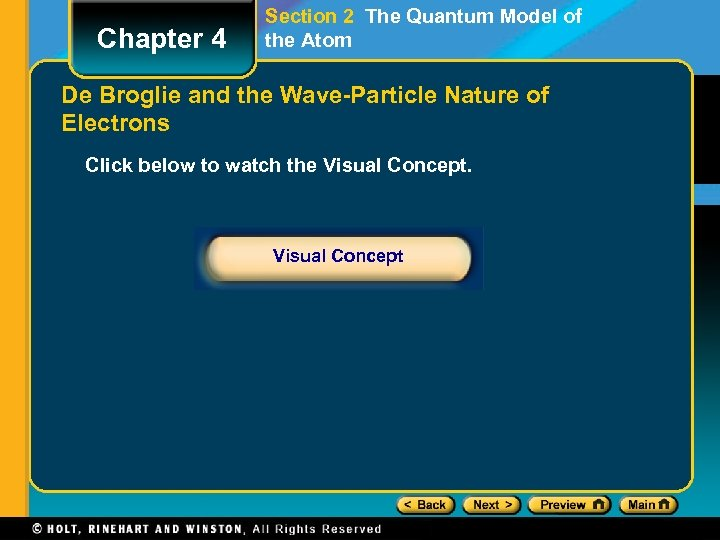Chapter 4 Section 2 The Quantum Model of the Atom De Broglie and the Wave-Particle Nature of Electrons Click below to watch the Visual Concept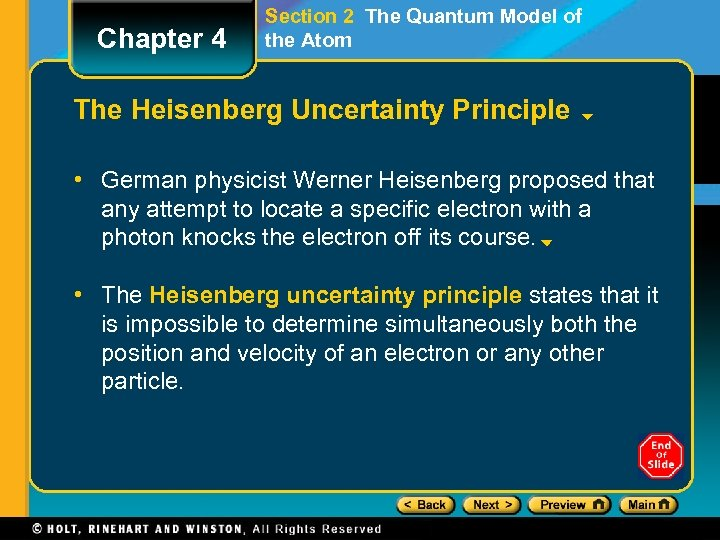Chapter 4 Section 2 The Quantum Model of the Atom The Heisenberg Uncertainty Principle • German physicist Werner Heisenberg proposed that any attempt to locate a specific electron with a photon knocks the electron off its course. • The Heisenberg uncertainty principle states that it is impossible to determine simultaneously both the position and velocity of an electron or any other particle.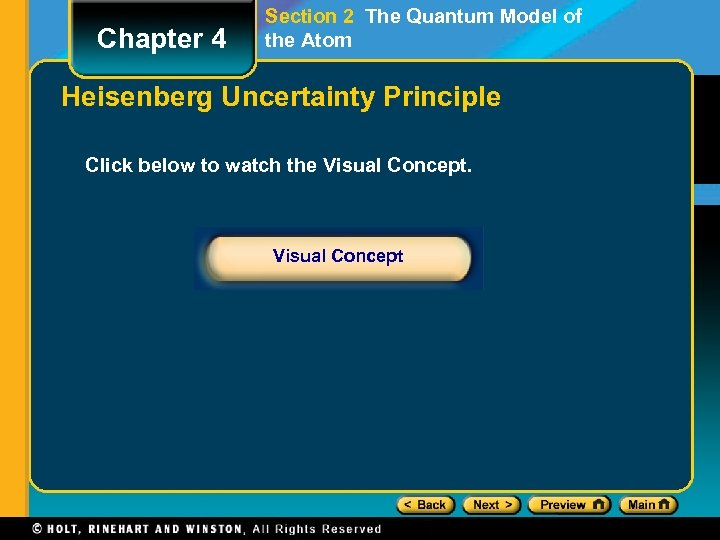Chapter 4 Section 2 The Quantum Model of the Atom Heisenberg Uncertainty Principle Click below to watch the Visual Concept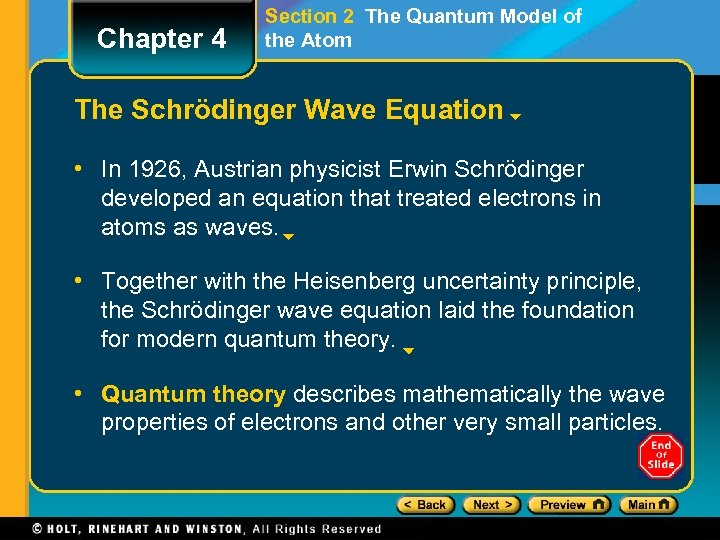Chapter 4 Section 2 The Quantum Model of the Atom The Schrödinger Wave Equation • In 1926, Austrian physicist Erwin Schrödinger developed an equation that treated electrons in atoms as waves. • Together with the Heisenberg uncertainty principle, the Schrödinger wave equation laid the foundation for modern quantum theory. • Quantum theory describes mathematically the wave properties of electrons and other very small particles.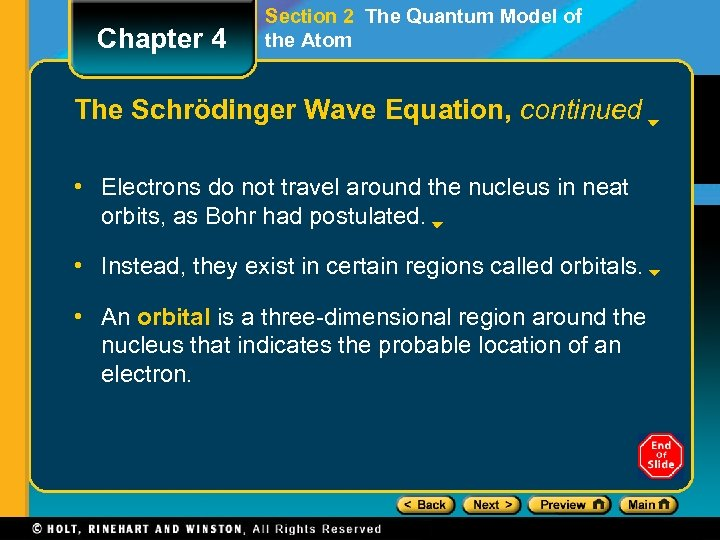Chapter 4 Section 2 The Quantum Model of the Atom The Schrödinger Wave Equation, continued • Electrons do not travel around the nucleus in neat orbits, as Bohr had postulated. • Instead, they exist in certain regions called orbitals. • An orbital is a three-dimensional region around the nucleus that indicates the probable location of an electron.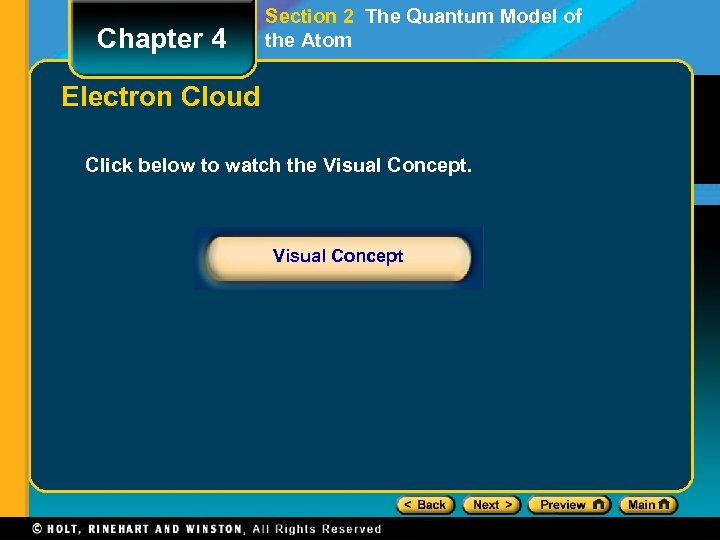Chapter 4 Section 2 The Quantum Model of the Atom Electron Cloud Click below to watch the Visual Concept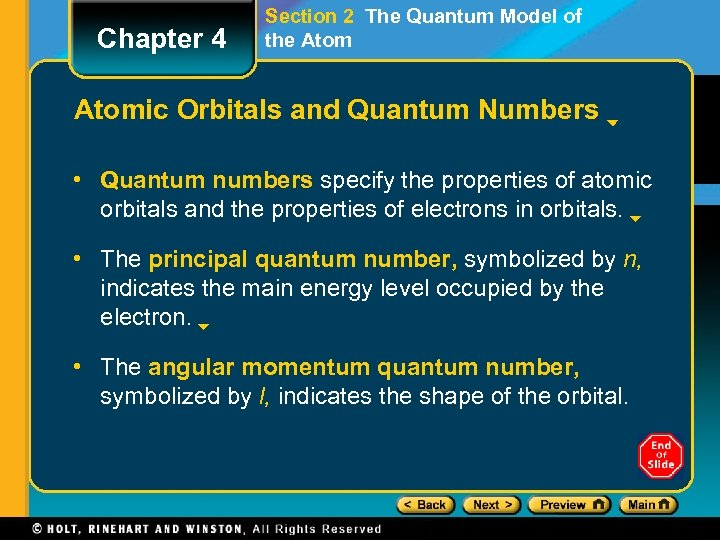Chapter 4 Section 2 The Quantum Model of the Atomic Orbitals and Quantum Numbers • Quantum numbers specify the properties of atomic orbitals and the properties of electrons in orbitals. • The principal quantum number, symbolized by n, indicates the main energy level occupied by the electron. • The angular momentum quantum number, symbolized by l, indicates the shape of the orbital.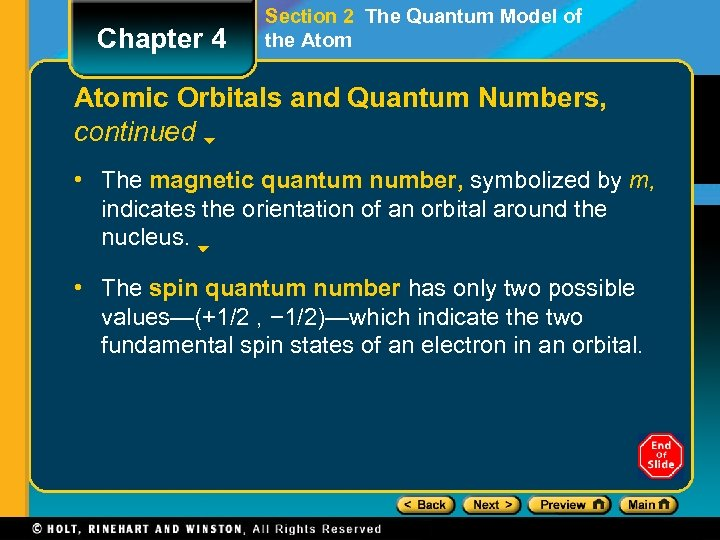Chapter 4 Section 2 The Quantum Model of the Atomic Orbitals and Quantum Numbers, continued • The magnetic quantum number, symbolized by m, indicates the orientation of an orbital around the nucleus. • The spin quantum number has only two possible values—(+1/2 , − 1/2)—which indicate the two fundamental spin states of an electron in an orbital.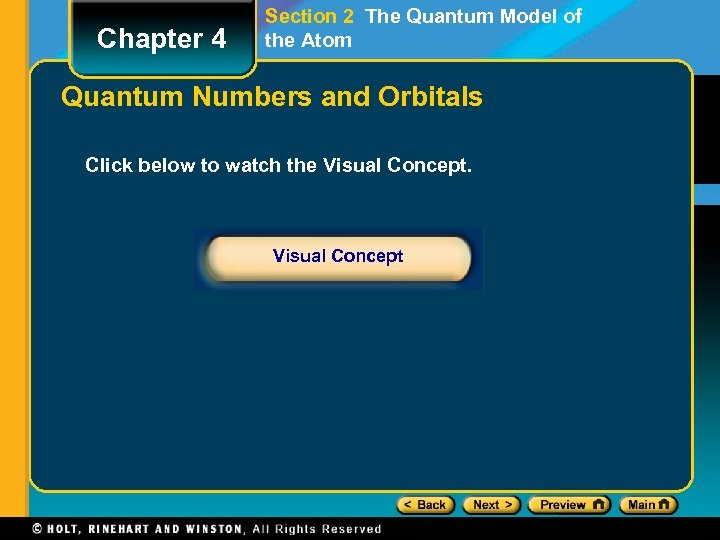Chapter 4 Section 2 The Quantum Model of the Atom Quantum Numbers and Orbitals Click below to watch the Visual Concept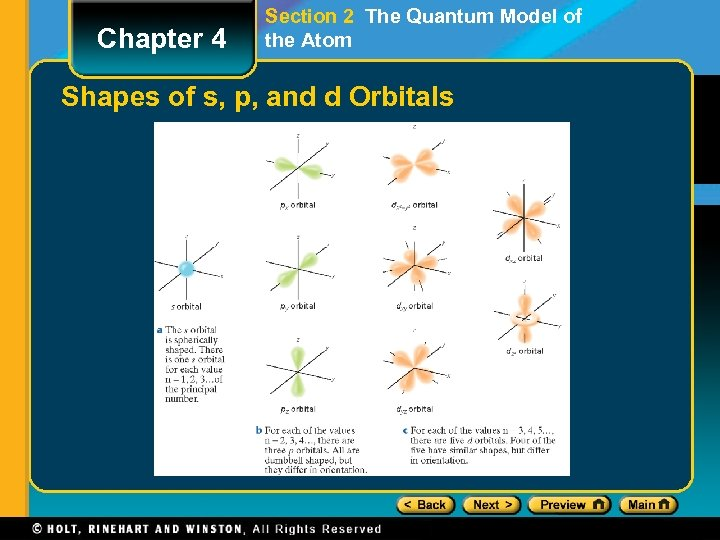Chapter 4 Section 2 The Quantum Model of the Atom Shapes of s, p, and d Orbitals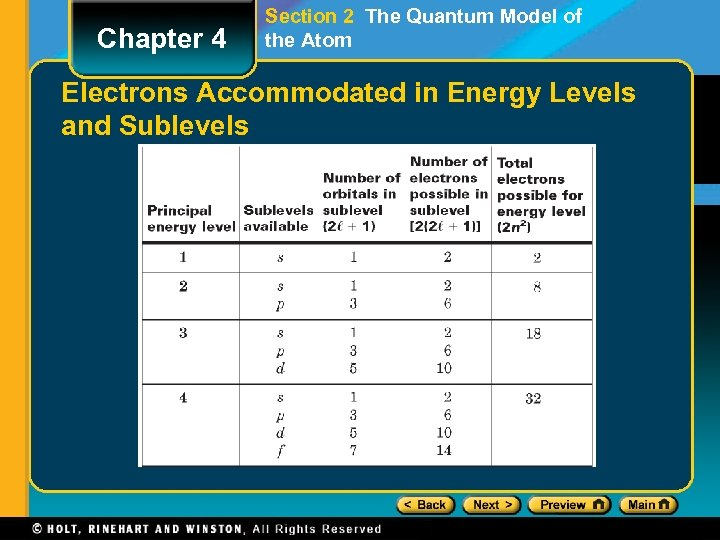Chapter 4 Section 2 The Quantum Model of the Atom Electrons Accommodated in Energy Levels and Sublevels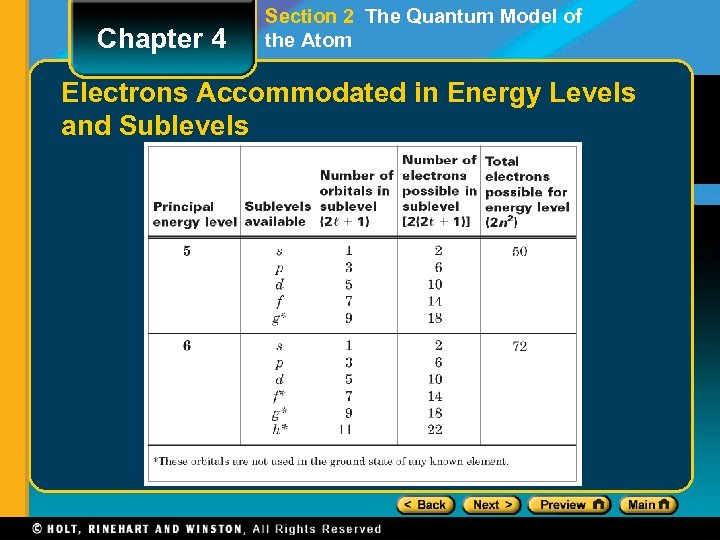Chapter 4 Section 2 The Quantum Model of the Atom Electrons Accommodated in Energy Levels and Sublevels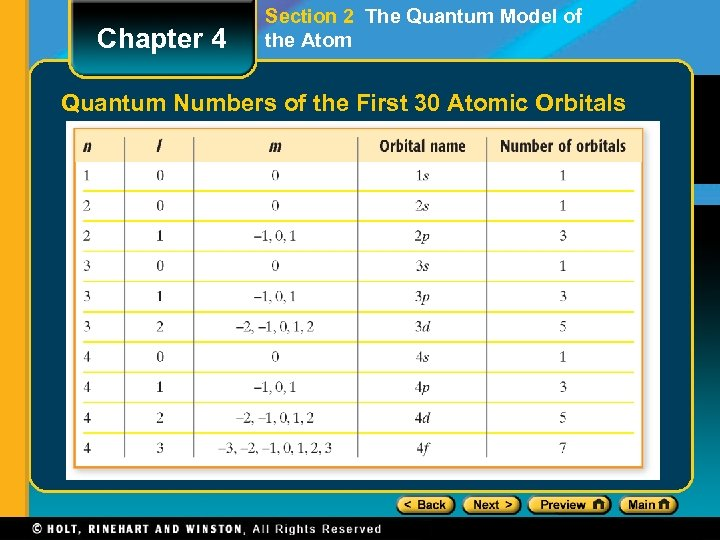Chapter 4 Section 2 The Quantum Model of the Atom Quantum Numbers of the First 30 Atomic Orbitals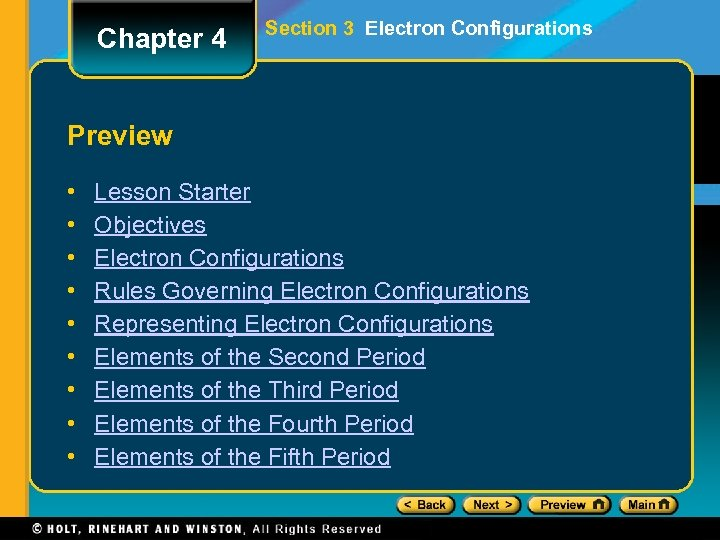Chapter 4 Section 3 Electron Configurations Preview • • • Lesson Starter Objectives Electron Configurations Rules Governing Electron Configurations Representing Electron Configurations Elements of the Second Period Elements of the Third Period Elements of the Fourth Period Elements of the Fifth Period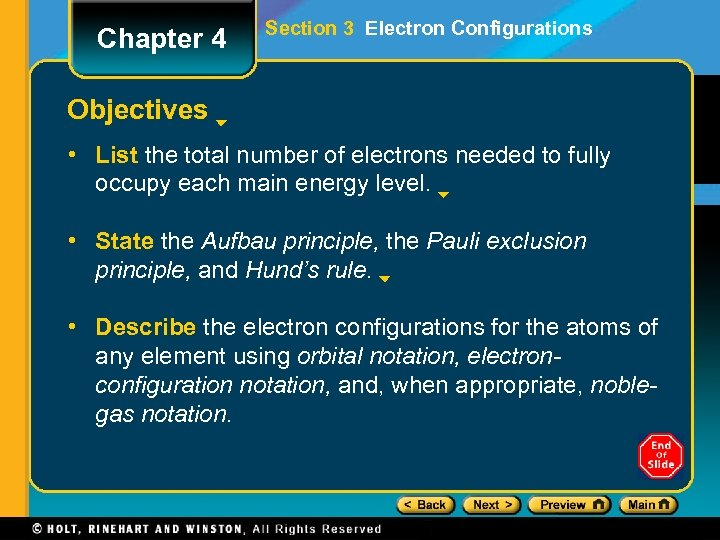Chapter 4 Section 3 Electron Configurations Objectives • List the total number of electrons needed to fully occupy each main energy level. • State the Aufbau principle, the Pauli exclusion principle, and Hund’s rule. • Describe the electron configurations for the atoms of any element using orbital notation, electronconfiguration notation, and, when appropriate, noblegas notation.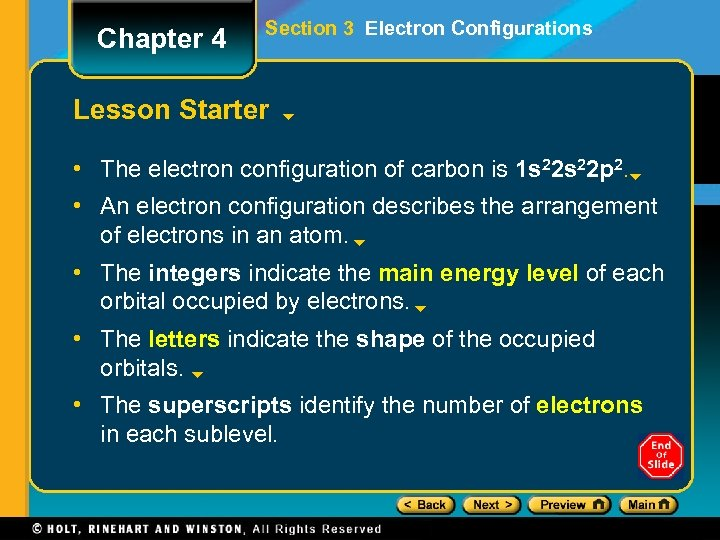Chapter 4 Section 3 Electron Configurations Lesson Starter • The electron configuration of carbon is 1 s 22 p 2. • An electron configuration describes the arrangement of electrons in an atom. • The integers indicate the main energy level of each orbital occupied by electrons. • The letters indicate the shape of the occupied orbitals. • The superscripts identify the number of electrons in each sublevel.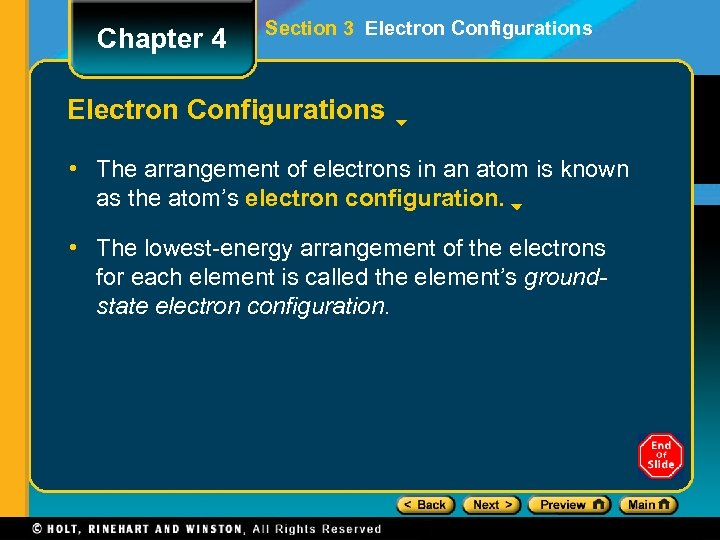Chapter 4 Section 3 Electron Configurations • The arrangement of electrons in an atom is known as the atom’s electron configuration. • The lowest-energy arrangement of the electrons for each element is called the element’s groundstate electron configuration.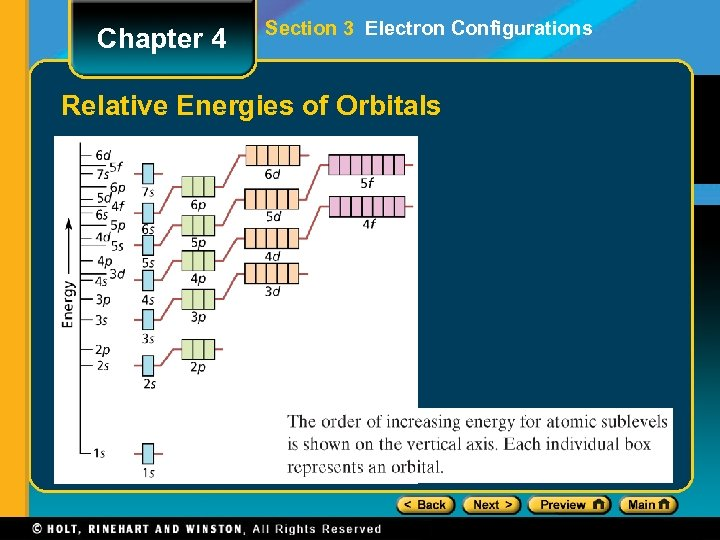Chapter 4 Section 3 Electron Configurations Relative Energies of Orbitals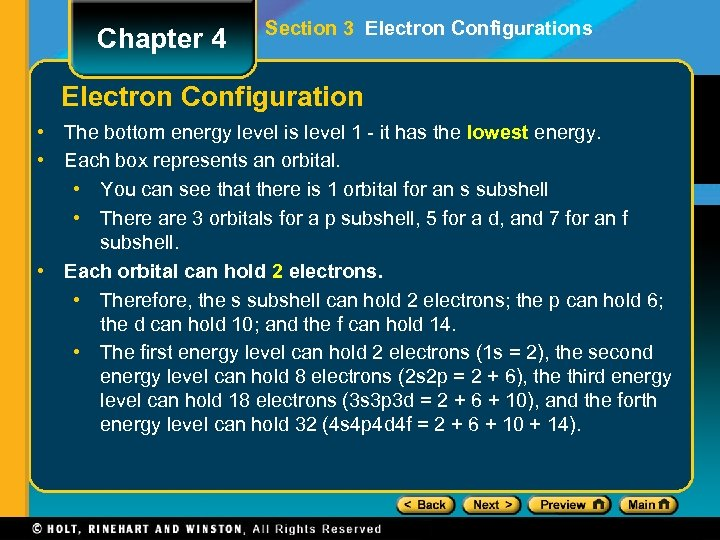Chapter 4 Section 3 Electron Configurations Electron Configuration • The bottom energy level is level 1 - it has the lowest energy. • Each box represents an orbital. • You can see that there is 1 orbital for an s subshell • There are 3 orbitals for a p subshell, 5 for a d, and 7 for an f subshell. • Each orbital can hold 2 electrons. • Therefore, the s subshell can hold 2 electrons; the p can hold 6; the d can hold 10; and the f can hold 14. • The first energy level can hold 2 electrons (1 s = 2), the second energy level can hold 8 electrons (2 s 2 p = 2 + 6), the third energy level can hold 18 electrons (3 s 3 p 3 d = 2 + 6 + 10), and the forth energy level can hold 32 (4 s 4 p 4 d 4 f = 2 + 6 + 10 + 14).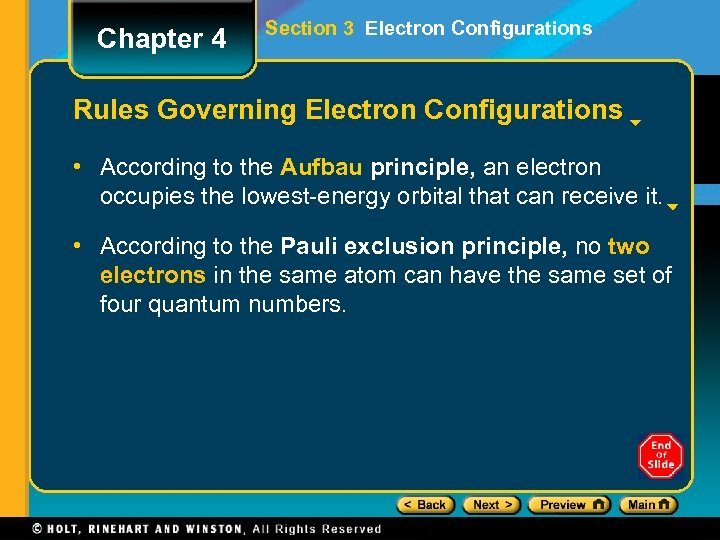Chapter 4 Section 3 Electron Configurations Rules Governing Electron Configurations • According to the Aufbau principle, an electron occupies the lowest-energy orbital that can receive it. • According to the Pauli exclusion principle, no two electrons in the same atom can have the same set of four quantum numbers.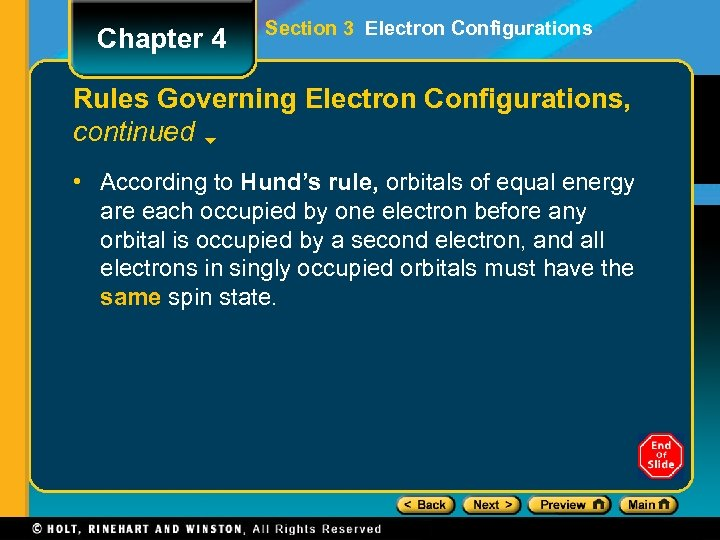Chapter 4 Section 3 Electron Configurations Rules Governing Electron Configurations, continued • According to Hund’s rule, orbitals of equal energy are each occupied by one electron before any orbital is occupied by a second electron, and all electrons in singly occupied orbitals must have the same spin state.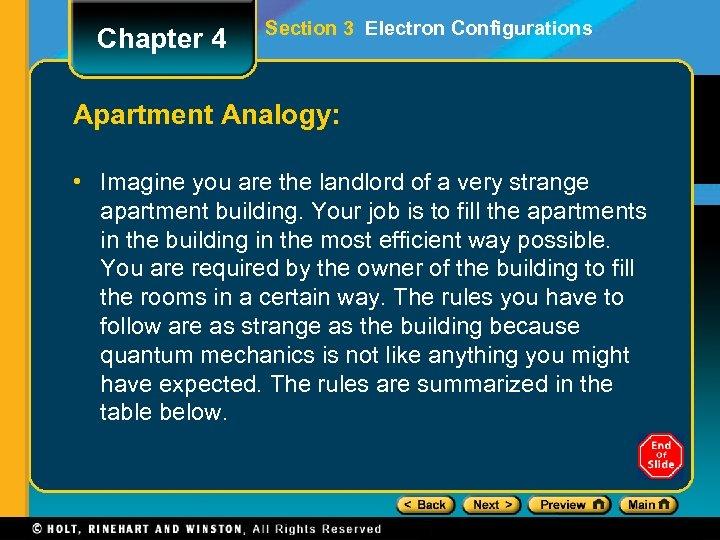Chapter 4 Section 3 Electron Configurations Apartment Analogy: • Imagine you are the landlord of a very strange apartment building. Your job is to fill the apartments in the building in the most efficient way possible. You are required by the owner of the building to fill the rooms in a certain way. The rules you have to follow are as strange as the building because quantum mechanics is not like anything you might have expected. The rules are summarized in the table below.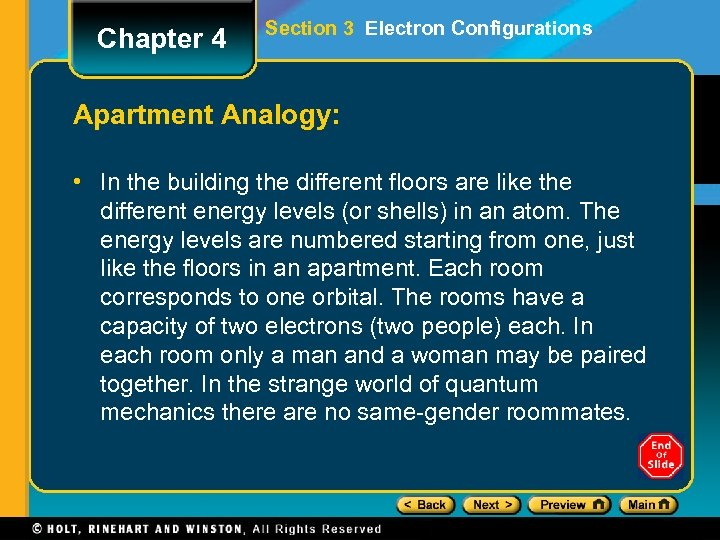Chapter 4 Section 3 Electron Configurations Apartment Analogy: • In the building the different floors are like the different energy levels (or shells) in an atom. The energy levels are numbered starting from one, just like the floors in an apartment. Each room corresponds to one orbital. The rooms have a capacity of two electrons (two people) each. In each room only a man and a woman may be paired together. In the strange world of quantum mechanics there are no same-gender roommates.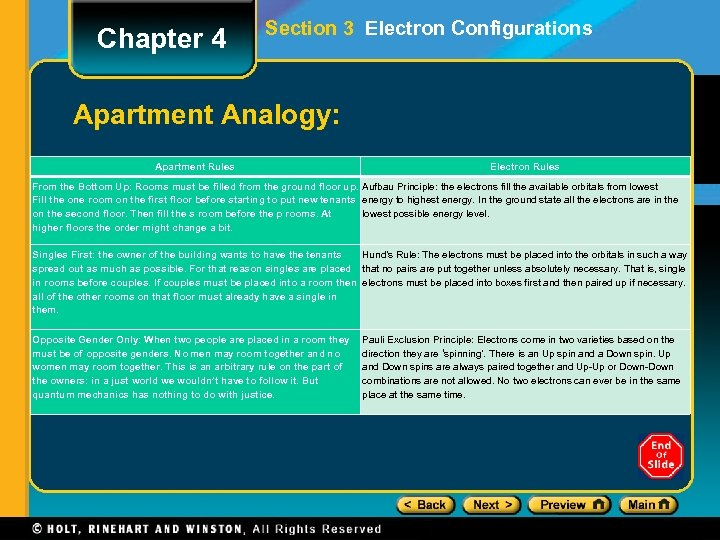Chapter 4 Section 3 Electron Configurations Apartment Analogy: Apartment Rules Electron Rules From the Bottom Up: Rooms must be filled from the ground floor up. Aufbau Principle: the electrons fill the available orbitals from lowest Fill the one room on the first floor before starting to put new tenants energy to highest energy. In the ground state all the electrons are in the on the second floor. Then fill the s room before the p rooms. At lowest possible energy level. higher floors the order might change a bit. Singles First: the owner of the building wants to have the tenants Hund’s Rule: The electrons must be placed into the orbitals in such a way spread out as much as possible. For that reason singles are placed that no pairs are put together unless absolutely necessary. That is, single in rooms before couples. If couples must be placed into a room then electrons must be placed into boxes first and then paired up if necessary. all of the other rooms on that floor must already have a single in them. Opposite Gender Only: When two people are placed in a room they must be of opposite genders. No men may room together and no women may room together. This is an arbitrary rule on the part of the owners: in a just world we wouldn’t have to follow it. But quantum mechanics has nothing to do with justice. Pauli Exclusion Principle: Electrons come in two varieties based on the direction they are ‘spinning’. There is an Up spin and a Down spin. Up and Down spins are always paired together and Up-Up or Down-Down combinations are not allowed. No two electrons can ever be in the same place at the same time.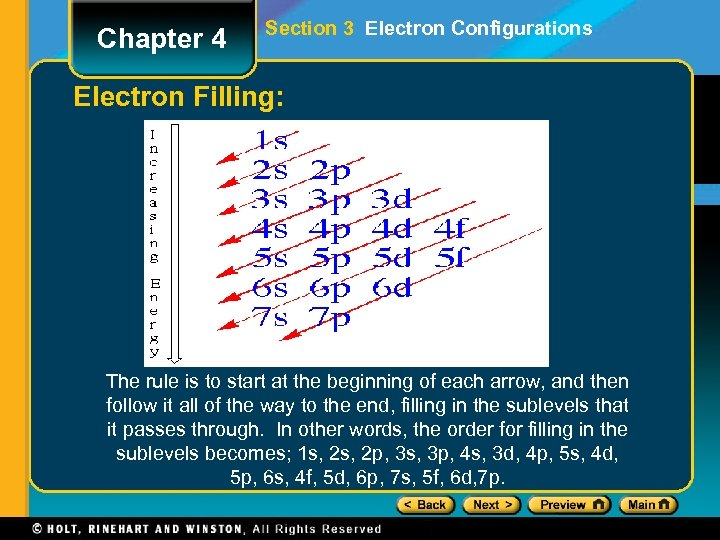Chapter 4 Section 3 Electron Configurations Electron Filling: The rule is to start at the beginning of each arrow, and then follow it all of the way to the end, filling in the sublevels that it passes through. In other words, the order for filling in the sublevels becomes; 1 s, 2 p, 3 s, 3 p, 4 s, 3 d, 4 p, 5 s, 4 d, 5 p, 6 s, 4 f, 5 d, 6 p, 7 s, 5 f, 6 d, 7 p.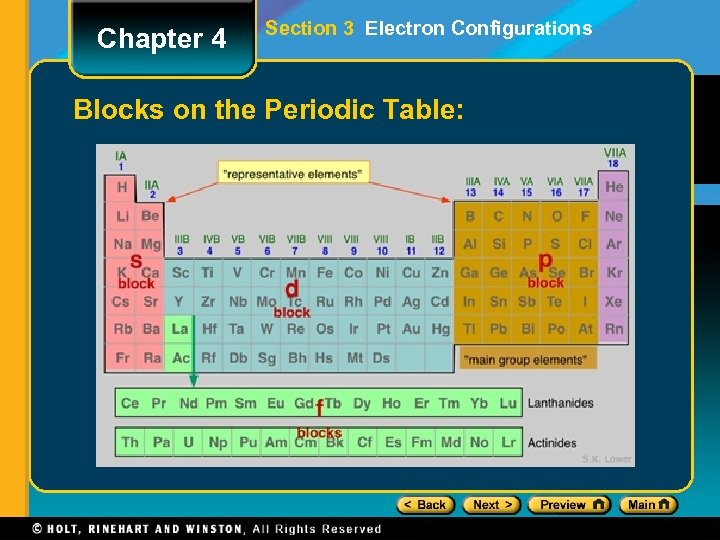Chapter 4 Section 3 Electron Configurations Blocks on the Periodic Table: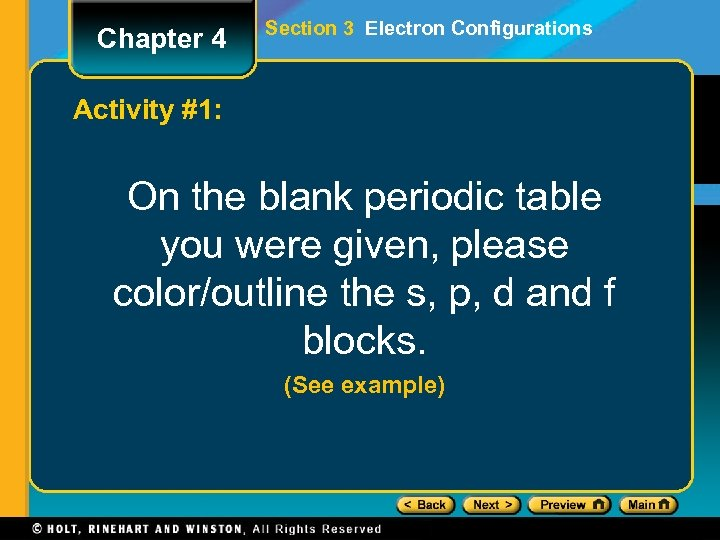Chapter 4 Section 3 Electron Configurations Activity #1: On the blank periodic table you were given, please color/outline the s, p, d and f blocks. (See example)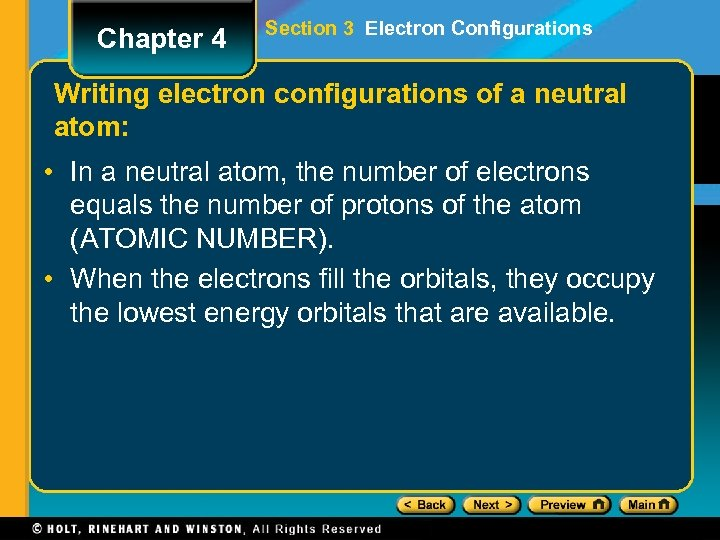Chapter 4 Section 3 Electron Configurations Writing electron configurations of a neutral atom: • In a neutral atom, the number of electrons equals the number of protons of the atom (ATOMIC NUMBER). • When the electrons fill the orbitals, they occupy the lowest energy orbitals that are available.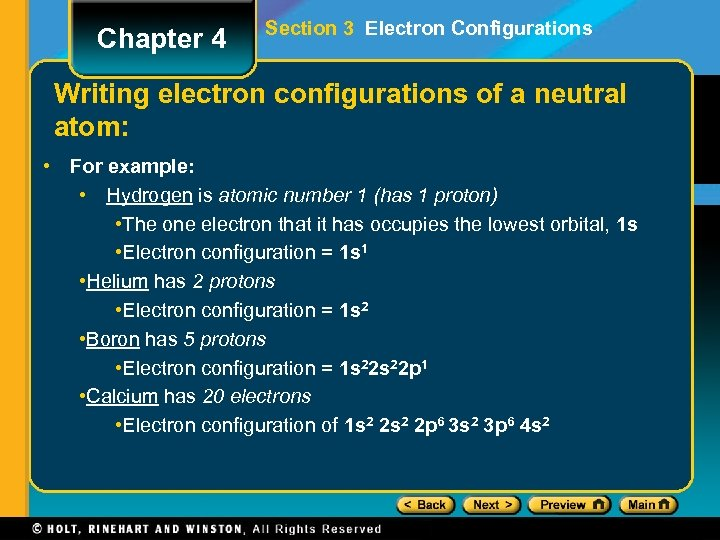Chapter 4 Section 3 Electron Configurations Writing electron configurations of a neutral atom: • For example: • Hydrogen is atomic number 1 (has 1 proton) • The one electron that it has occupies the lowest orbital, 1 s • Electron configuration = 1 s 1 • Helium has 2 protons • Electron configuration = 1 s 2 • Boron has 5 protons • Electron configuration = 1 s 22 p 1 • Calcium has 20 electrons • Electron configuration of 1 s 2 2 p 6 3 s 2 3 p 6 4 s 2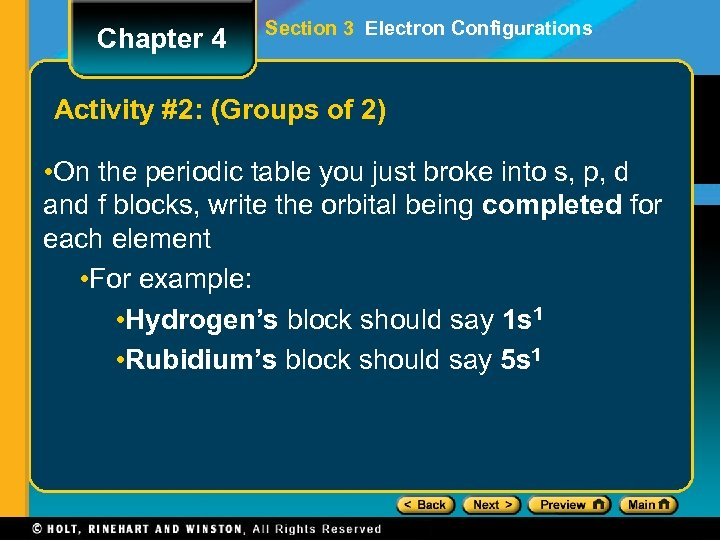Chapter 4 Section 3 Electron Configurations Activity #2: (Groups of 2) • On the periodic table you just broke into s, p, d and f blocks, write the orbital being completed for each element • For example: • Hydrogen’s block should say 1 s 1 • Rubidium’s block should say 5 s 1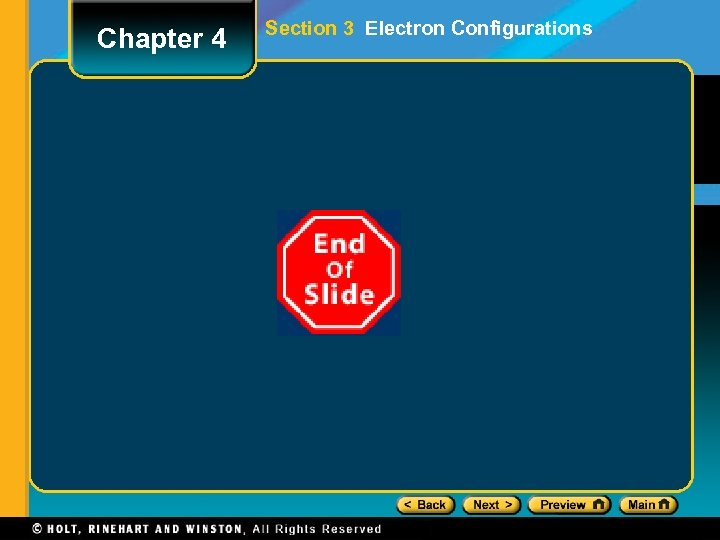Chapter 4 Section 3 Electron Configurations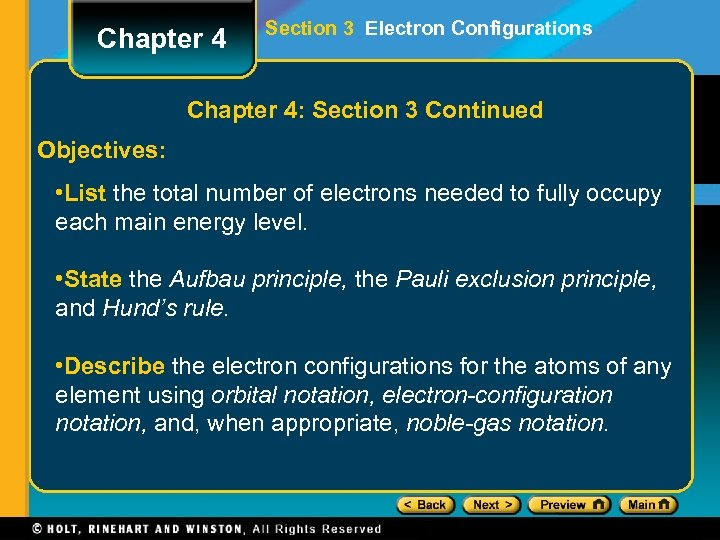Chapter 4 Section 3 Electron Configurations Chapter 4: Section 3 Continued Objectives: • List the total number of electrons needed to fully occupy each main energy level. • State the Aufbau principle, the Pauli exclusion principle, and Hund’s rule. • Describe the electron configurations for the atoms of any element using orbital notation, electron-configuration notation, and, when appropriate, noble-gas notation.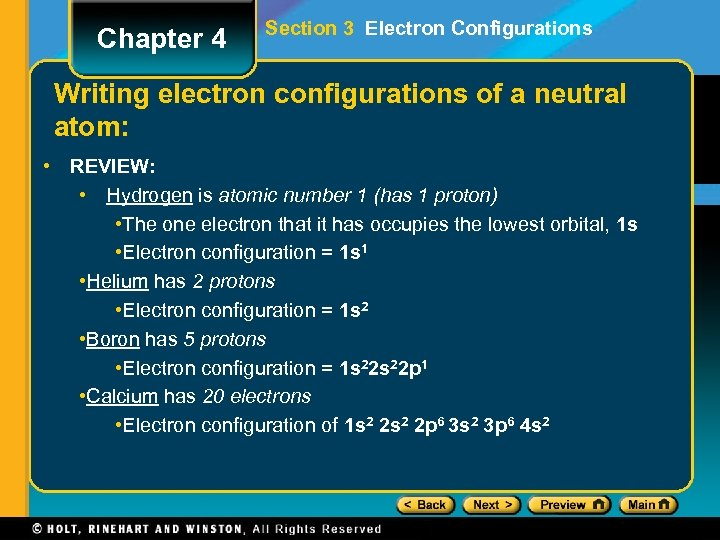Chapter 4 Section 3 Electron Configurations Writing electron configurations of a neutral atom: • REVIEW: • Hydrogen is atomic number 1 (has 1 proton) • The one electron that it has occupies the lowest orbital, 1 s • Electron configuration = 1 s 1 • Helium has 2 protons • Electron configuration = 1 s 2 • Boron has 5 protons • Electron configuration = 1 s 22 p 1 • Calcium has 20 electrons • Electron configuration of 1 s 2 2 p 6 3 s 2 3 p 6 4 s 2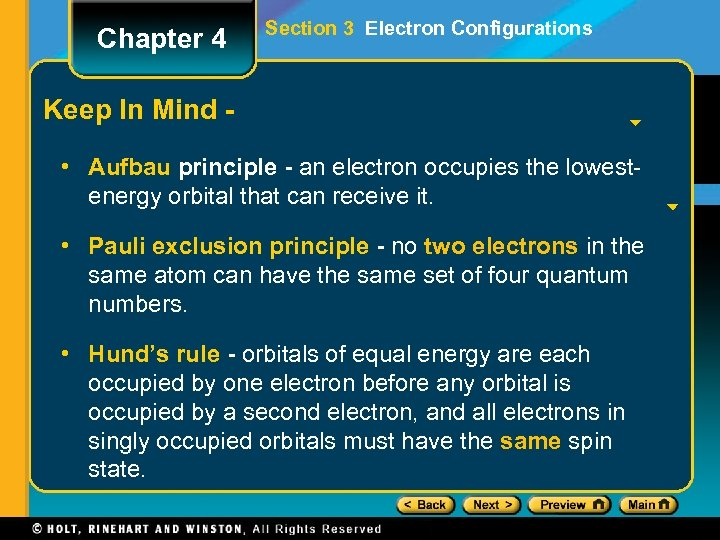Chapter 4 Section 3 Electron Configurations Keep In Mind • Aufbau principle - an electron occupies the lowestenergy orbital that can receive it. • Pauli exclusion principle - no two electrons in the same atom can have the same set of four quantum numbers. • Hund’s rule - orbitals of equal energy are each occupied by one electron before any orbital is occupied by a second electron, and all electrons in singly occupied orbitals must have the same spin state.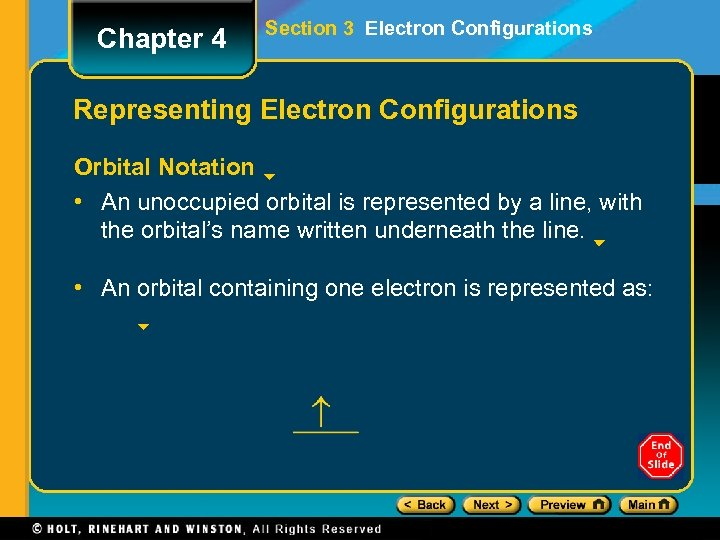Chapter 4 Section 3 Electron Configurations Representing Electron Configurations Orbital Notation • An unoccupied orbital is represented by a line, with the orbital’s name written underneath the line. • An orbital containing one electron is represented as: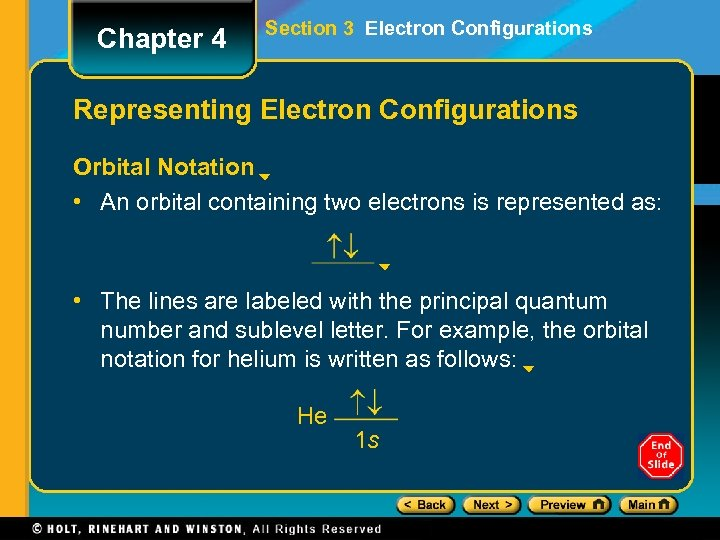Chapter 4 Section 3 Electron Configurations Representing Electron Configurations Orbital Notation • An orbital containing two electrons is represented as: • The lines are labeled with the principal quantum number and sublevel letter. For example, the orbital notation for helium is written as follows: He 1 sChapter 4 Section 3 Electron Configurations Drawing Orbital Notation Click below to watch the Visual Concept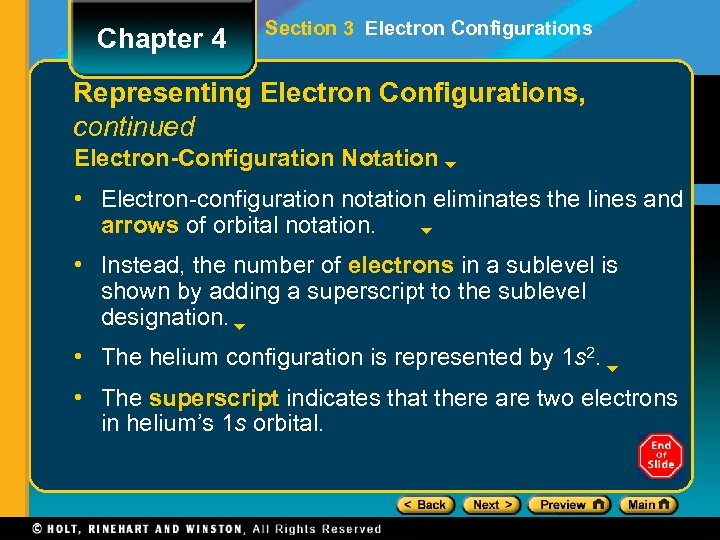Chapter 4 Section 3 Electron Configurations Representing Electron Configurations, continued Electron-Configuration Notation • Electron-configuration notation eliminates the lines and arrows of orbital notation. • Instead, the number of electrons in a sublevel is shown by adding a superscript to the sublevel designation. • The helium configuration is represented by 1 s 2. • The superscript indicates that there are two electrons in helium’s 1 s orbital.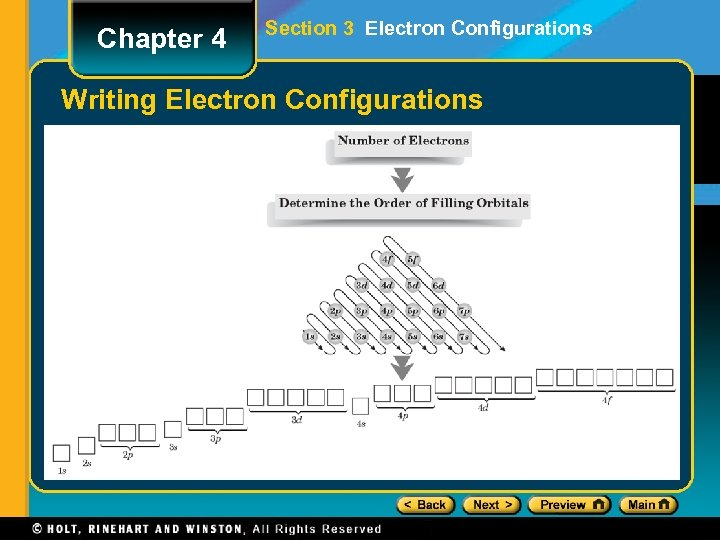Chapter 4 Section 3 Electron Configurations Writing Electron Configurations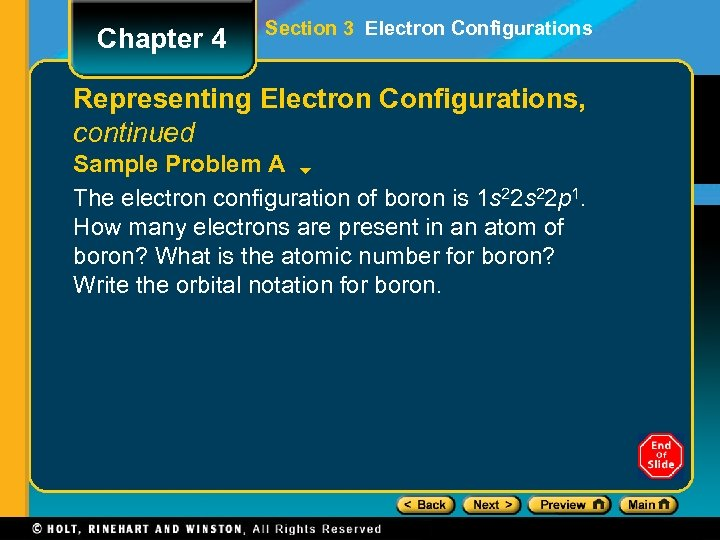Chapter 4 Section 3 Electron Configurations Representing Electron Configurations, continued Sample Problem A The electron configuration of boron is 1 s 22 p 1. How many electrons are present in an atom of boron? What is the atomic number for boron? Write the orbital notation for boron.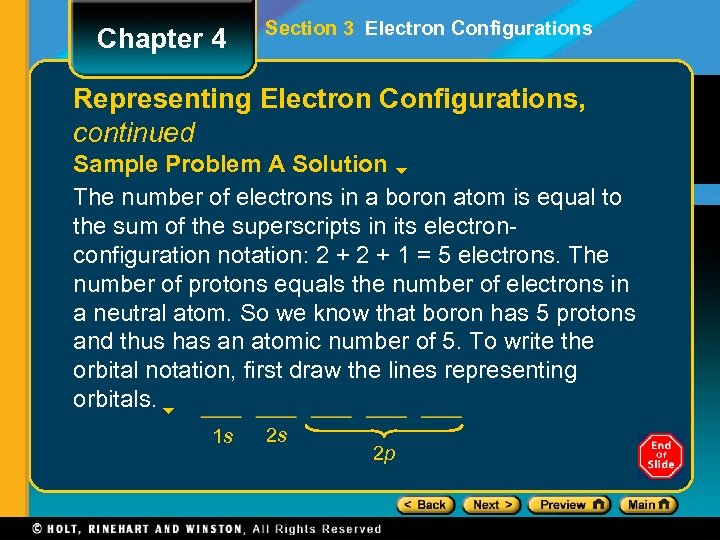Chapter 4 Section 3 Electron Configurations Representing Electron Configurations, continued Sample Problem A Solution The number of electrons in a boron atom is equal to the sum of the superscripts in its electronconfiguration notation: 2 + 1 = 5 electrons. The number of protons equals the number of electrons in a neutral atom. So we know that boron has 5 protons and thus has an atomic number of 5. To write the orbital notation, first draw the lines representing orbitals. 1 s 2 s 2 p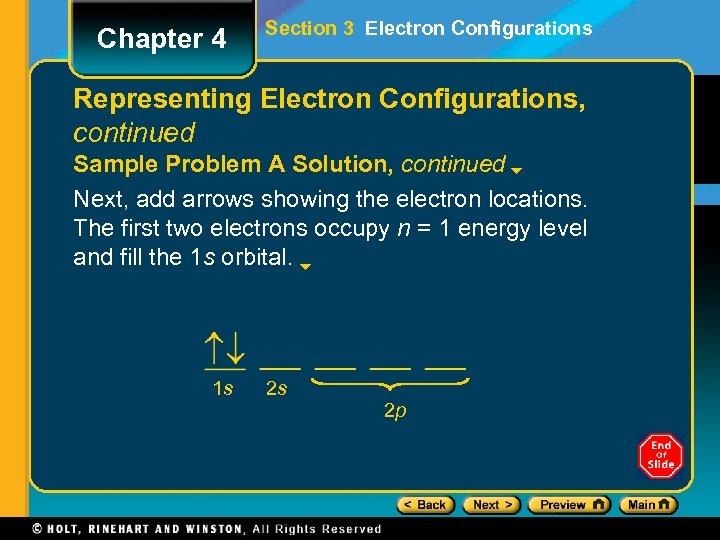Chapter 4 Section 3 Electron Configurations Representing Electron Configurations, continued Sample Problem A Solution, continued Next, add arrows showing the electron locations. The first two electrons occupy n = 1 energy level and fill the 1 s orbital. 1 s 2 s 2 p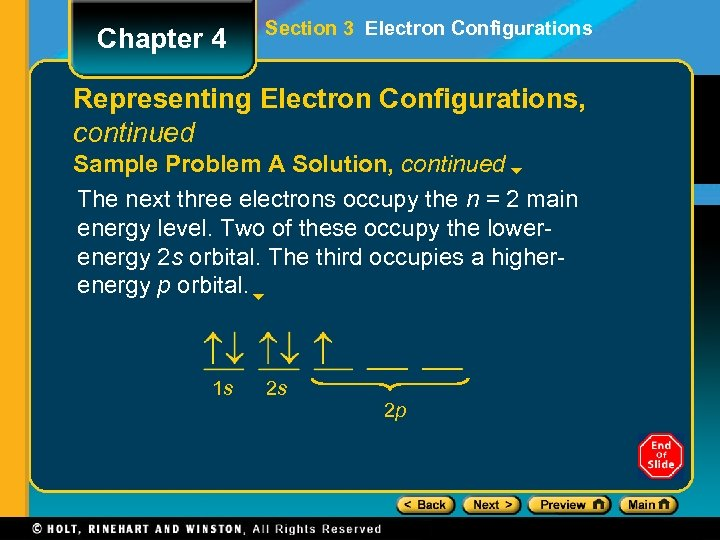Chapter 4 Section 3 Electron Configurations Representing Electron Configurations, continued Sample Problem A Solution, continued The next three electrons occupy the n = 2 main energy level. Two of these occupy the lowerenergy 2 s orbital. The third occupies a higherenergy p orbital. 1 s 2 s 2 p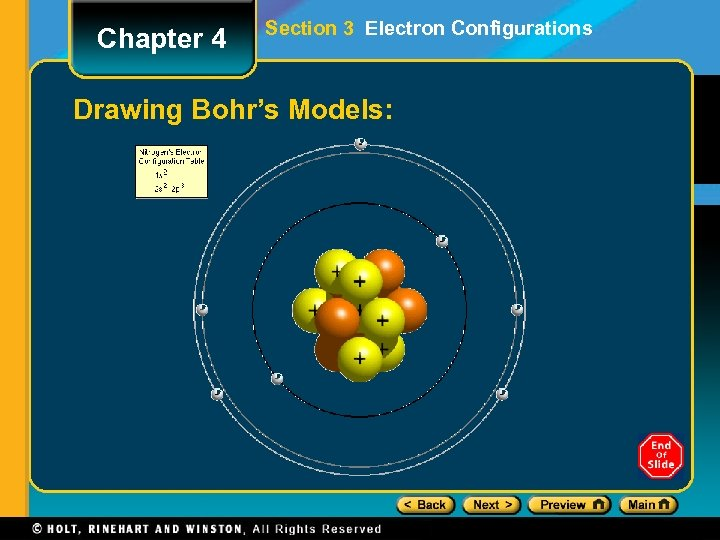Chapter 4 Section 3 Electron Configurations Drawing Bohr’s Models: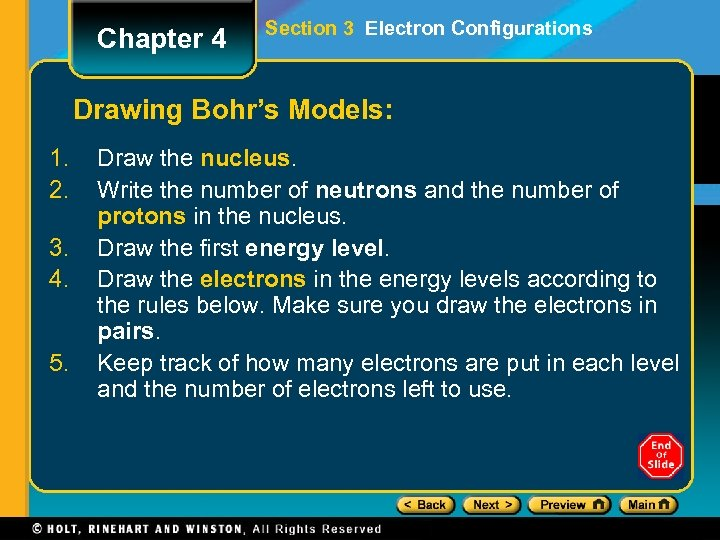Chapter 4 Section 3 Electron Configurations Drawing Bohr’s Models: 1. 2. 3. 4. 5. Draw the nucleus. Write the number of neutrons and the number of protons in the nucleus. Draw the first energy level. Draw the electrons in the energy levels according to the rules below. Make sure you draw the electrons in pairs. Keep track of how many electrons are put in each level and the number of electrons left to use.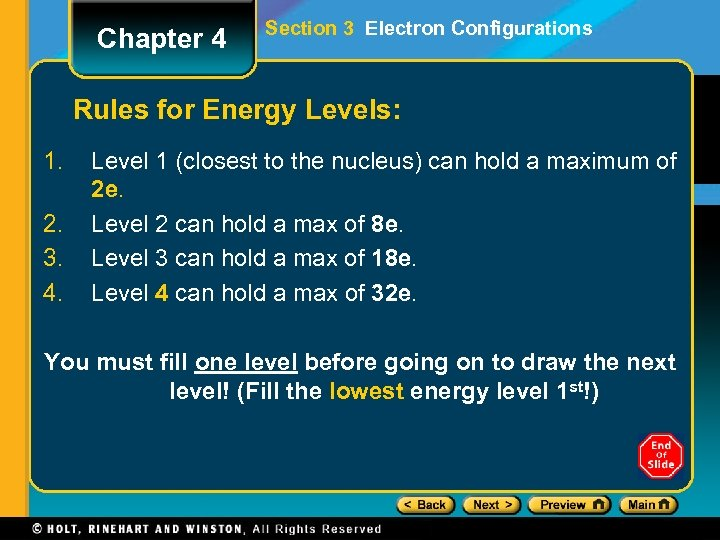Chapter 4 Section 3 Electron Configurations Rules for Energy Levels: 1. 2. 3. 4. Level 1 (closest to the nucleus) can hold a maximum of 2 e. Level 2 can hold a max of 8 e. Level 3 can hold a max of 18 e. Level 4 can hold a max of 32 e. You must fill one level before going on to draw the next level! (Fill the lowest energy level 1 st!)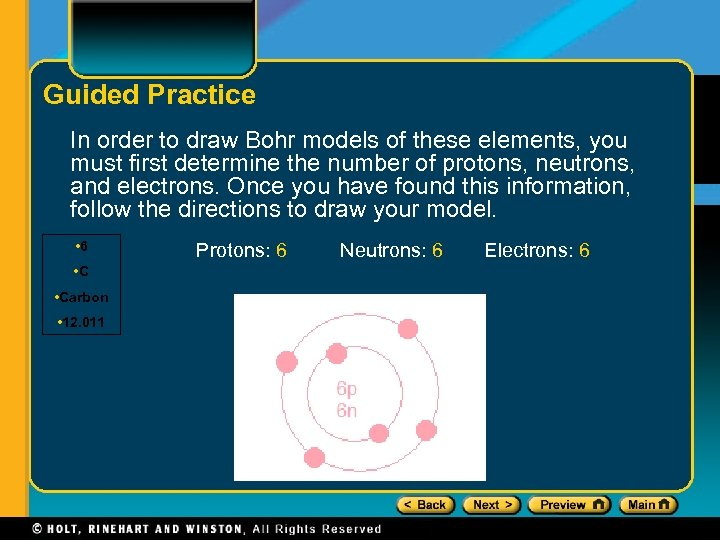Guided Practice In order to draw Bohr models of these elements, you must first determine the number of protons, neutrons, and electrons. Once you have found this information, follow the directions to draw your model. • 6 • Carbon • 12. 011 Protons: 6 Neutrons: 6 Electrons: 6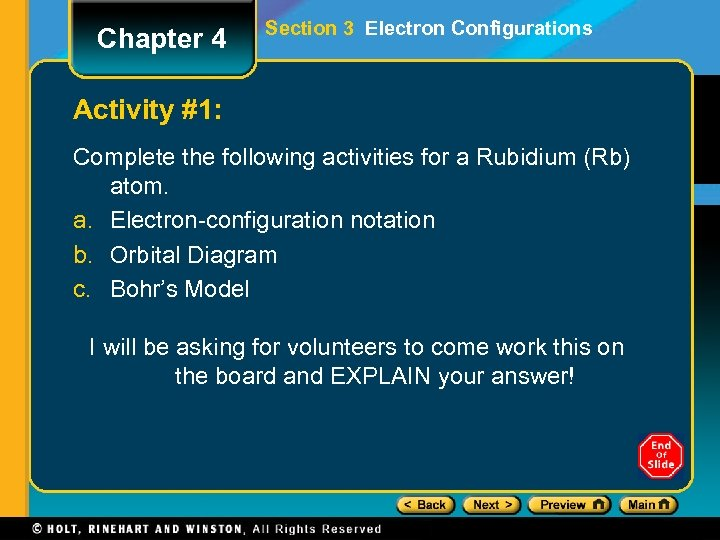Chapter 4 Section 3 Electron Configurations Activity #1: Complete the following activities for a Rubidium (Rb) atom. a. Electron-configuration notation b. Orbital Diagram c. Bohr’s Model I will be asking for volunteers to come work this on the board and EXPLAIN your answer!Chapter 4 Section 3 Electron Configurations Classwork for Today/Beginning of Period Tomorrow: Electron Configuration Resource/Practice Worksheet Please complete as much of this as possible today… I will give you a few minutes in class to finish this tomorrow (due to Nine Weeks Exams). Once your time is up, I will call on people to write their answers on the board and EXPLAIN.Chapter 4 Section 3 Electron Configurations FINISHING CHAPTER 4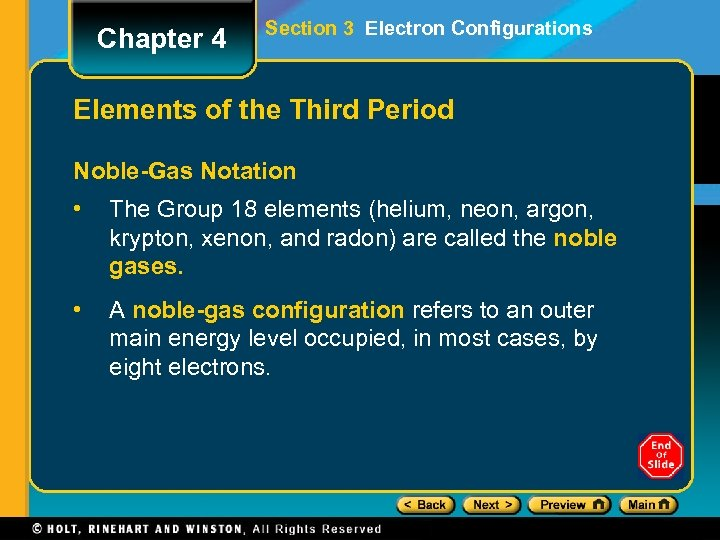Chapter 4 Section 3 Electron Configurations Elements of the Third Period Noble-Gas Notation • The Group 18 elements (helium, neon, argon, krypton, xenon, and radon) are called the noble gases. • A noble-gas configuration refers to an outer main energy level occupied, in most cases, by eight electrons.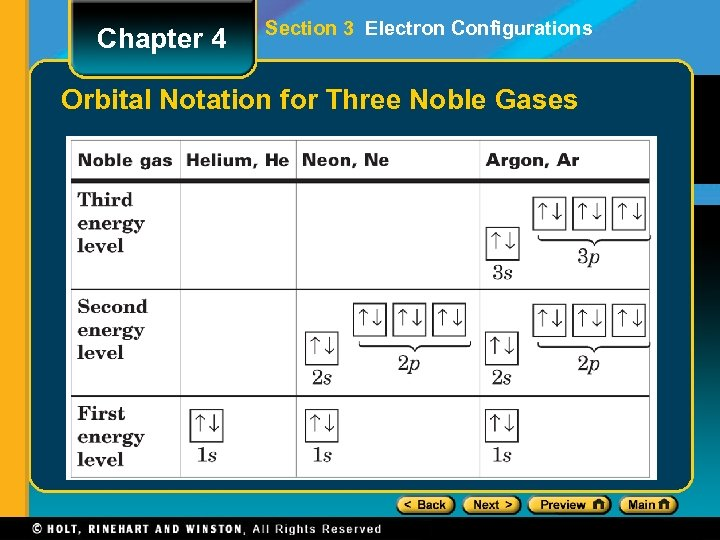Chapter 4 Section 3 Electron Configurations Orbital Notation for Three Noble GasesChapter 4 Section 3 Electron Configurations Noble-Gas Notation Click below to watch the Visual Concept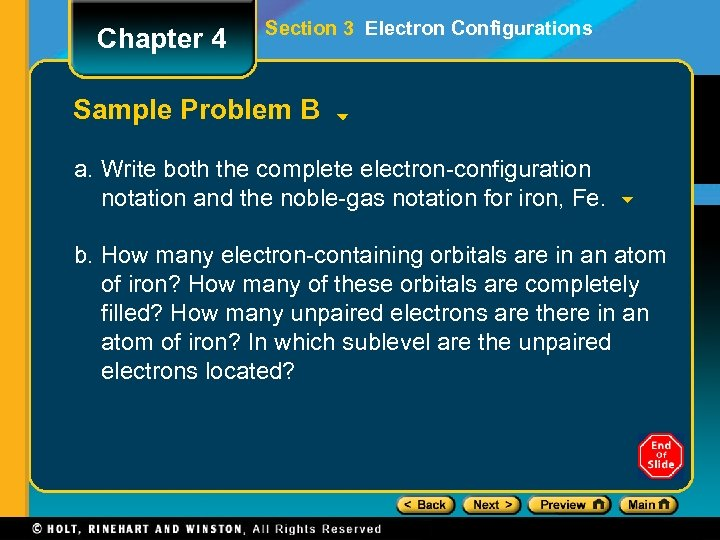Chapter 4 Section 3 Electron Configurations Sample Problem B a. Write both the complete electron-configuration notation and the noble-gas notation for iron, Fe. b. How many electron-containing orbitals are in an atom of iron? How many of these orbitals are completely filled? How many unpaired electrons are there in an atom of iron? In which sublevel are the unpaired electrons located?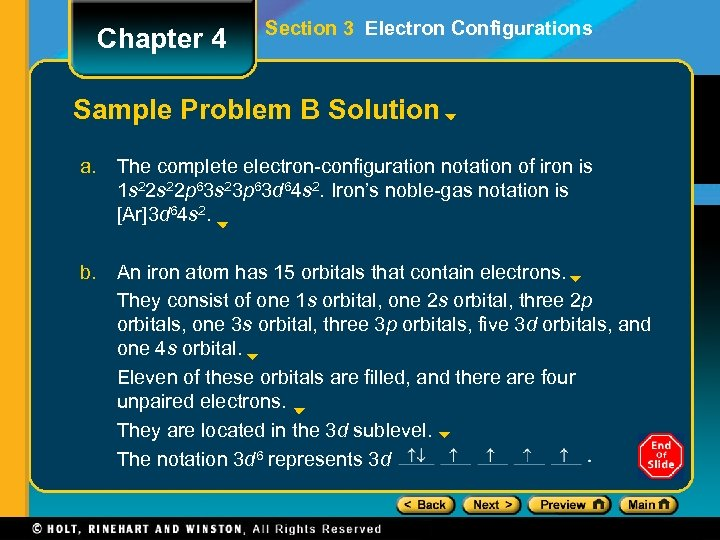Chapter 4 Section 3 Electron Configurations Sample Problem B Solution a. The complete electron-configuration notation of iron is 1 s 22 p 63 s 23 p 63 d 64 s 2. Iron’s noble-gas notation is [Ar]3 d 64 s 2. b. An iron atom has 15 orbitals that contain electrons. They consist of one 1 s orbital, one 2 s orbital, three 2 p orbitals, one 3 s orbital, three 3 p orbitals, five 3 d orbitals, and one 4 s orbital. Eleven of these orbitals are filled, and there are four unpaired electrons. They are located in the 3 d sublevel. The notation 3 d 6 represents 3 dEnd of Chapter 4 Show